• 一、文本处理流程 文本清洗：html标签与转义字符、多余空格、根据需要清除邮箱、账号、网址、手机号、作者信息等信息 预处理：去除停用词、加载自定义词库（实体词库、垂直领域词库）、分词 特征提取：...

一、文本处理流程

1. 文本清洗：html标签与转义字符、多余空格、根据需要清除邮箱、账号、网址、手机号、作者信息等信息

2. 预处理：去除停用词、加载自定义词库（实体词库、垂直领域词库）、分词

3. 特征提取：关键词、实体词

4. 建模：文本分类、文本聚类、情感分析、标签提取

5. 优化：停用词库与自定义词库清洗与丰富、文本标签清洗、模型调整

6. 效果评估：满足线上使用要求，准确率、速度

7. 上线部署：部署api接口

二、NLP算法

• 深度学习在自然语言处理当中，除了在分类问题上能够取得较好效果外（如单选问题：情感分类、文本分类、正确答案分类问题等），在信息抽取上，尤其是在元组抽取上基本上是一塌糊涂，在工业场景下很难达到实用水准
• 目前各种评测集大多是人为标注的，人为标注的大多为干净环境下的较为规范的文本，而且省略了真实生产环节中的多个环节。在评测环节中达到的诸多state-of-art方法，在真实应用场景下泛化能力很差，大多仅仅是为了刷榜而刷榜。
• 目前关于知识图谱的构建环节中，数据大多数都还是来自于结构化数据，半结构化信息抽取次之，非结构化数据抽取最少。半结构化信息抽取，即表格信息抽取最为危险，一个单元格错误很有可能导致所有数据都出现错误。非结构化抽取中，实体识别和实体关系识别难度相当大
• 工业场景下命名实体识别，标配的BILSTM+CRF实际上只是辅助手段，工业界还是以领域实体字典匹配为主，大厂中往往在后者有很大的用户日志，这种日志包括大量的实体信息。因此，生产环节中的实体识别工作中，基础性词性的构建和扩展工作显得尤为重要。
• 目前关于知识图谱推理问题，严格意义上不属于推理的范畴，最多只能相当于是知识补全问题，如评测中的知识推理任务，是三元组补全问题。
• 目前舆情分析还是处于初级阶段。目前舆情分析还停留在以表层计量为主，配以浅层句子级情感分析和主题挖掘技术的分析。对于深层次事件演化以及对象级情感分析依旧还处于初级阶段。
• Bert本质上仅仅是个编码器，是word2vec的升级版而已，不是无所不能，仅仅是编码能力强，向量表示上语义更为丰富，然而大多人都装糊涂。
• 学界和业界最大的区别在于，学界以探索前沿为目的，提新概念，然后搭个草图就结束，目光并不长远，打完这一战就不知道下一战打什么，下一战该去哪里打，什么时候打，或者打一枪换个阵地再打。而业界，往往面临着生存问题，需要考虑实际问题，还是以解决实际问题为主，因此没必要把学界的那一套理念融入到生产环节中，要根据实际情况制定自己的方法
• 利用结构化数据，尤其是百科类infobox数据，采集下来，存入到Neo4j图数据库中，就称自己建立了知识图谱的做法是伪知识图谱做法。基于这类知识图谱，再搞个简单的问答系统，就标榜自己是基于知识图谱的智能问答，实际上很肤浅。
• 知识图谱不是结构化知识的可视化（不是两个点几条边）那么简单，那叫知识的可视化，不是知识图谱。知识图谱的核心在于知识的图谱化，特点在于知识的表示方法和图谱存储结构，前者决定了知识的抽象表示维度，后者决定了知识运行的可行性，图算法(图遍历、联通图、最短路径)。基于图谱存储结构，进行知识的游走，进行知识表征和未知知识的预测。
• 物以稀为贵，大家都能获取到的知识，往往价值都很低。知识图谱也是这样，只有做专门性的具有数据壁垒的知识图谱，才能带来商业价值。
• 目前智能问答，大多都是人工智障，通用型的闲聊型问答大多是个智障，多轮对话缺失答非所问等问题层出不穷。垂直性的问答才是出路，但真正用心做的太少，大多都是处于demo级别。
• 大多数微信自然语言处理软文实际上都不可不看，纯属浪费时间。尤其是在对内容的分析上，大多是抓语料，调包统计词频，提取关键词，调包情感分析，做柱状图，做折线图，做主题词云，分析方法上千篇一律。应该从根本上去做方法上的创新，这样才能有营养，从根本上来说才能有营养可言。文本分析应该从浅层分析走向深层分析，更好地挖掘文本的语义信息。
• 目前百科类知识图谱的构建工作有很多，重复性的工作不少。基于开放类百科知识图谱的数据获取接口有复旦等开放出来，可以应用到基本的概念下实体查询，实体属性查询等，但目前仅仅只能做到一度。
• 基于知识图谱的问答目前的难点在于两个方面，1）多度也称为多跳问题，如姚明的老婆是谁，可以走14条回答，但姚明的老婆的女儿是谁则回答不出来，这种本质上是实体与属性以及实体与实体关系的分类问题。2）多轮问答问题。多轮分成两种，一种是指代补全问答， 如前一句问北京的天气，后者省略“的天气”这一词，而只说“北京”，这个需要进行意图判定并准确加载相应的问答槽。另一种是追问式多轮问答，典型的在天气查询或者酒店预订等垂直性问答任务上。大家要抓住这两个方面去做。
• 关系挖掘是信息抽取的重要里程碑，理解了实体与实体、实体与属性、属性与属性、实体与事件、事件与事件的关系是解决真正语义理解的基础，但目前，这方面，在工业界实际运用中，特定领域中模板的性能要比深度学习多得多，学界大多采用端到端模型进行实验，在这方面还难以超越模版性能。

1、TF-IDF

1）原理：词频-逆文档频率；一种用于信息检索与文本挖掘的常用加权技术；一种统计方法，用以评估一个字词对于一个文件集或一个语料库中的其中一份文件的重要程度，字词的重要性随着它在文件中出现的次数成正比增加，但同时会随着它在语料库中出现的频率成反比下降。

2）主要思想：如果某个单词在一篇文章中出现的频率TF高，并且在其他文章中很少出现，则认为此词或者短语具有很好的类别区分能力，适合用来分类。

3）公式：

TF=在某一文本中词条w出现的次数/该文本中所有的词条数目

IDF=log(语料库的文档总数/(包含词条w的文档数+1))，避免分母为0

TF-IDF=TF*IDF

4）优缺点：实现简单，容易理解，但未考虑词语语义信息，无法处理一词多义与一义多词

5）应用：搜索引擎，关键词提取，文本相似性计算，文本摘要

6）python调参：

提取关键词

keywords = jieba.analyse.extract_tags(sentence, topK=10, withWeight=False, allowPOS=())    # 主要调参指定词性的词

提取特征向量

tfidf = TfidfVectorizer(ngram_range=(1, 2), min_df=1, max_df=0.9, token_pattern=r"(?u)\b\w+\b", stop_words=stopwords, max_features=1000)
'''
ngram_range=(1, 2) : 词组长度为1和2
min_df : 忽略出现频率小于1的词
max_df : 忽略在百分之九十以上的文本中出现过的词
token_pattern=r"(?u)\b\w+\b" : 包含单字
stop_words : 停用词表
max_features=1000: 最大特征向量维度
'''

2、Textrank

1）原理：将每个句子看成一个节点，若两个句子之间有相似性，认为对应的两个节点之间有一个无向有权边，权值是相似度。通过pagerank算法（两个假设：数量假设，页面A被其他网页链接越多，页面A越重要；质量假设，质量越高的页面链接指向页面A，页面A越重要）计算得到的重要性最高的若干句子可以当作摘要。PageRank主要用于对在线搜索结果中的网页进行排序

PageRank的核心公式是PageRank值的计算公式。公式如下：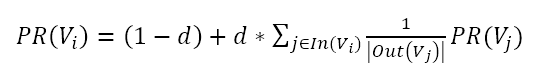其中，PR(Vi)表示结点Vi的rank值，In(Vi)表示结点Vi的前驱结点集合，Out(Vj)表示结点Vj的后继结点集合。
这个公式来自于《统计学习方法》，等号右边的平滑项（通过某种处理，避免一些突变的畸形值，尽可能接近实际情况）不是（1-d），而是(1-d)/n。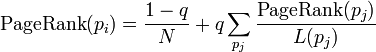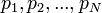是被研究的页面，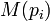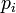链入页面的数量，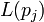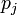链出页面的数量，而N是所有页面的数量。

在文本自动摘要的案例中，TextRank和PageRank的相似之处在于：

• 用句子代替网页

• 任意两个句子的相似性等价于网页转换概率

• 相似性得分存储在一个方形矩阵中，类似于PageRank的矩阵M

不过公式有些小的差别，那就是用句子的相似度类比于网页转移概率，用归一化的句子相似度代替了PageRank中相等的转移概率，这意味着在TextRank中，所有节点的转移概率不会完全相等。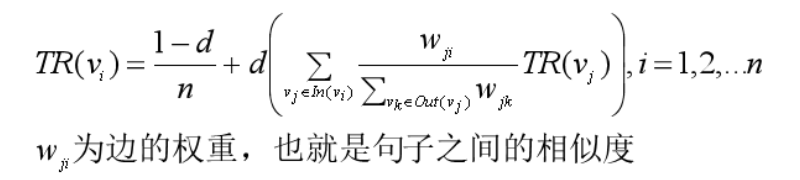TextRank算法是一种抽取式的无监督的文本摘要方法。让我们看一下我们将遵循的TextRank算法的流程：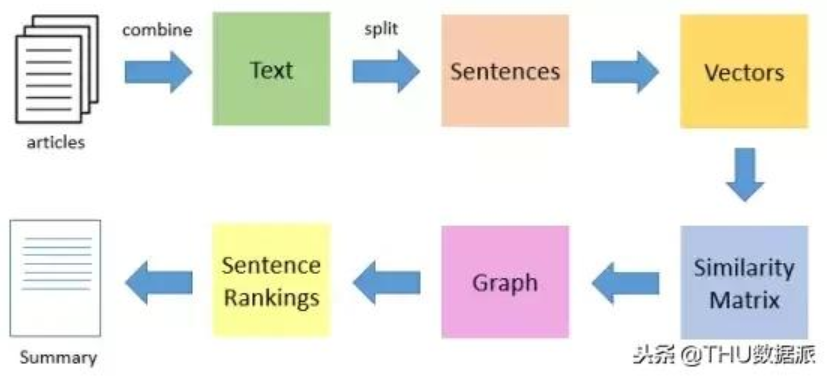• 停用词库
• 语料库
• 自定义分词词库
• 人物库
• 垂直领域词库

4、知识图谱

• 三元组
• NEO4教程：Neo4j是一个世界领先的开源图形数据库。 它是由Neo技术使用Java语言完全开发的。
• CQL代表Cypher查询语言。 像Oracle数据库具有查询语言SQL，Neo4j具有CQL作为查询语言。

Neo4j CQL：

• 它是Neo4j图形数据库的查询语言。

• 它是一种声明性模式匹配语言

• 它遵循SQL语法。

• 它的语法是非常简单且人性化、可读的格式。

5、预训练模型

语言模型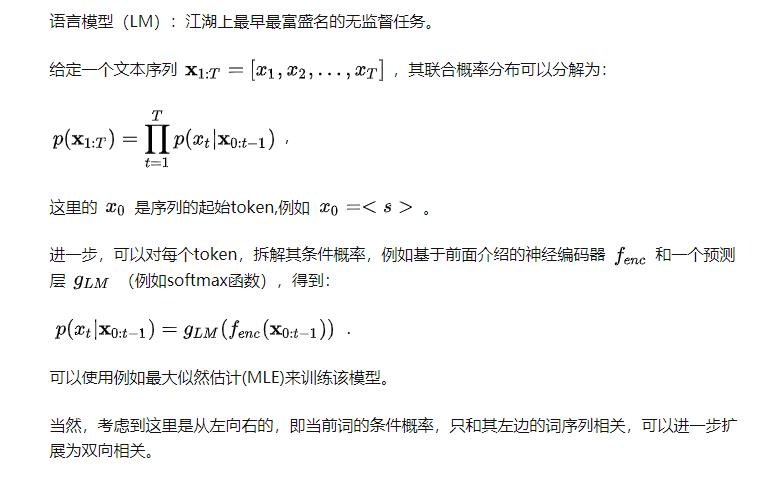预训练模型(Pre-trained Models,PTMs)

深度学习时代，为了充分训练深层模型参数防止过拟合，通常需要更多标注数据喂养。在NLP领域，标注数据更是一个昂贵资源。PTMs从大量无标注数据中进行预训练使许多NLP任务获得显著的性能提升。总的来看，预训练模型PTMs的优势包括：

1. 在庞大的无标注数据上进行预训练可以获取更通用的语言表示，并有利于下游任务；
2. 为模型提供了一个更好的初始化参数，在目标任务上具备更好的泛化性能、并加速收敛；
3. 是一种有效的正则化手段，避免在小数据集上过拟合（一个随机初始化的深层模型容易对小数据集过拟合）；

词嵌入是自然语言处理（NLP）中语言模型与表征学习技术的统称。概念上而言，它是指把一个维数为所有词的数量的高维空间嵌入到一个维数低得多的连续向量空间中，每个单词或词组被映射为实数域上的向量，这也是分布式表示：向量的每一维度都没有实际意义，而整体代表一个具体概念。

分布式表示相较于传统的独热编码（one-hot）表示具备更强的表示能力，而独热编码存在维度灾难和语义鸿沟（不能进行相似度计算）等问题。传统的分布式表示方法，如矩阵分解（SVD/LSA）、LDA等均是根据全局语料进行训练，是机器学习时代的产物。PTMs也属于分布式表示的范畴。

PTMs两大范式：「浅层词嵌入」和「预训练编码器」。

浅层词嵌入（ Non-Contextual Embeddings）：即词向量，其主要特点是学习到的是上下文独立的静态词嵌入，其主要代表如下。这一类词嵌入通常采取浅层网络进行训练，而应用于下游任务时，整个模型的其余部分仍需要从头开始学习。因此，对于这一范式的PTMs没有必要采取深层神经网络进行训练，采取浅层网络加速训练也可以产生好的词嵌入。

• NNLM
• word2vec（CBOW、Skip-Gram）
• Glove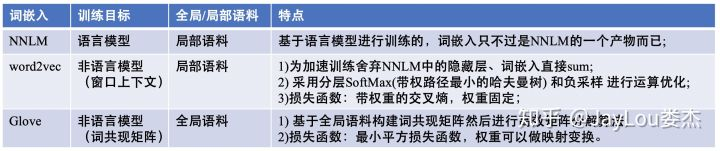浅层词嵌入的主要缺陷为：

• 词嵌入与上下文无关，每个单词的嵌入向量始终是相同，因此不能解决一词多义的问题。
• 通常会出现OOV问题（out of vocabulary，未登录词），为了解决这个问题，相关文献提出了字符级表示或sub-word表示，如CharCNN 、FastText和 Byte-Pair Encoding 。

预训练编码器（Contextual Embeddings：主要目的是通过一个预训练的编码器能够输出上下文相关的词向量，解决一词多义的问题。这一类预训练编码器输出的向量称之为「上下文相关的词嵌入」。这一类「预训练编码器」范式的PTMs主要代表有ELMOGPT-1BERTXLNet等。

• CNN（序列模型）：TEXTCNN，设定窗口，然后对窗口内的若干词进行卷积操作，得到这个窗口内的词的嵌入表示
• RNN（序列模型）：基于LSTM/GRU的，基于单向（从左向右）或者双向的RNN模型，对整个序列进行建模，得到每个词的语境下的嵌入表示
• ELMO
• BERT
• GPT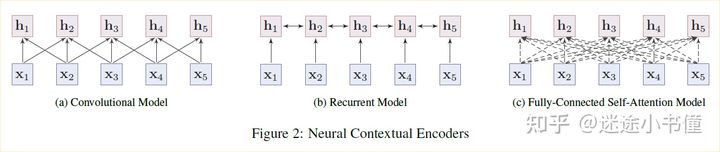上图中，(a)里面的卷积网络，可以认为窗口为3，例如 h2 由 x1、x2、x3 决定。(b)里面的 h2 由 h1 ，h3  和 x2 决定，具体可以使用LSTM, GRU等网络结构。(c)有点复杂，可以结合Transformer网络结构来理解。

分析：

序列模型：局部性偏见，难于捕捉长距离交互；但是容易训练，并在若干NLP任务上取得了不错的结果；

非序列模型：（代表是Transformer)更强大，使用的参数更多，可以更好的覆盖长距离依赖的问题；但是，需要大规模训练语料（可以是unlabel的！），容易在中小规模数据上过拟合。

当然，Transformer已经是目前几年的研究热点，不单单在NLP领域，在CV领域和speech领域也越来越引起重视，俨然成为了“中流砥柱”！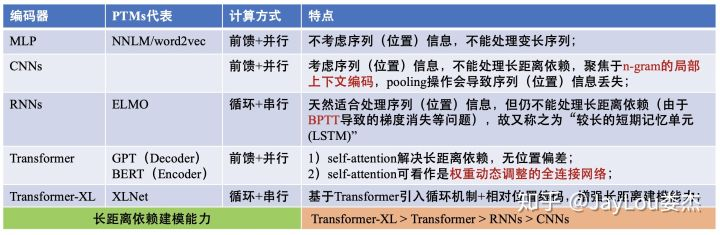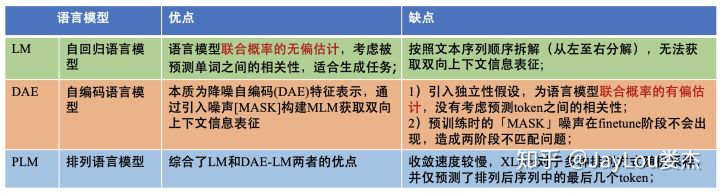三、参考文献

1. 实话实说：中文自然语言处理的N个真实情况

2. NLP中预训练模型的综述I

3. PTMs：NLP预训练模型的全面总结

4. 2020年3月18日，邱锡鹏老师发表了关于NLP预训练模型的综述《Pre-trained Models for Natural Language Processing: A Survey》，这是一篇全面的综述，系统地对PTMs进行了归纳分类

展开全文自然语言处理 nlp 机器学习 人工智能
• 自然语言处理-基于预训练模型的方法》笔记 文章目录《自然语言处理-基于预训练模型的方法》笔记@[toc]〇.写在前面一、绪论(一) NLP 任务体系I.任务层级II.任务类别III.研究层次(二) 预训练的时代二、NLP 基础(一) ...

《自然语言处理-基于预训练模型的方法》笔记

〇.写在前面

本笔记为哈工大“《自然语言处理-基于预训练模型的方法》——车万翔 郭江 崔一鸣 著 2021 年 7 月第一版” 的笔记，记录比较详细。不过，仍然强烈建议诸君购买原书进行学习！ 因为本笔记只是笔记，很多地方只是总结性的！

在阅读本笔记前，可能需要您具备一定的深度学习基础和代码能力基础，对于比较欠缺的同学，我给出了一定的学习指示与推荐，包括代码学习的材料以及理论学习的材料。不过无论如何，本笔记都需要一定的神经网络知识基础。详细请看本笔记第四章。

本笔记除了记录了书中知识点之外，还对书中的少量错误进行了修正，同时还进行了少量的扩展。

当然，本人才疏学浅，如有错误在所难免，恳请指正交流，不胜感激，嘤鸣求友！

in Markdown Homepage in HTML in PDF, 关于使用哪种格式，请看这里

Email : zenmoore@yeah.net, zenmoore@buaa.edu.cn

一、绪论

(一) NLP 任务体系

I.任务层级

1. 资源建设：语言学知识库 (词典、规则库)；语料库。

词典：也称 Thesaurus, 可以提供音韵、句法、语义解释、词汇关系、上下位同反义等

2. 基础任务：分词，词性标注，句法分析、句义分析等

3. 应用任务：信息抽取、情感分析、问答系统、机器翻译、对话系统等

4. 应用系统：教育、医疗、司法、金融、机器人等

II.任务类别

1. 回归问题：输出为连续数值
2. 分类问题
3. 匹配问题：判断关系
4. 解析问题：标注、词间关系
5. 生成问题

III.研究层次

1. 形式：符号
2. 语义：符号+实
3. 推理：符号+实+知 (常识知识、世界知识、领域知识)
4. 语用：符号+实+知+环境

(二) 预训练的时代

预训练+精调范式，预训练说是无监督并不准确，因为下一词预测这一个预训练任务仍然有监督，应该说成是自监督学习。

二、NLP 基础

(一) 文本表示

I.独热向量

1. one-hot vector
2. 可以引进额外特征表示共同语义 (如 WordNet 同义词等),转为特征工程

II.分布式表示

• 分布式语义假设 : 利用上下文进行语义建模

如使用共现词频(共现矩阵)： M = ( C ( w , c ) ) w ∈ V , c ∈ V \pmb{M}=(C(w,c))_{w\in\mathbb{V}, c\in{\mathbb{V}}}

C ( w , c ) C(w, c) 表示 词汇 w 与上下文词汇 c 共同出现的频次。
但存在直接使用存在 1.高频词问题 2.高阶关系 3.稀疏性问题

1. 点互信息 PMI : P M I = l o g 2 P ( w , c ) P ( w ) P ( c ) PMI=log_2{\frac{P(w, c)}{P(w)P(c)}} , 解决高频词问题

2. 正点互信息 PPMI : P P M I = m a x ( P M I ( w , c ) , 0 ) PPMI=max(PMI(w, c), 0) , 解决低共现频负 PMI 的不稳定性 (大方差).

3. TF-IDF : 解决高频词问题

4. 奇异值分解 SVD : M = U Σ V T ( U T U = V T V = I ) M=U\Sigma V^T (U^TU=V^TV=I) , 使用截断奇异值分解近似 M M (即取 d 个最大的奇异值)，矩阵 U U 的每一行即对应词的 d 维向量表示，该表示一般连续低维稠密。由于正交性，可以认为不同的维度的潜在语义相互独立。因此，这种方法也叫做 潜在语义分析 LSA. 相应地， Σ V T \Sigma V^T 的每一列也可以作为上下文的向量表示。解决了高阶关系问题和稀疏性问题。

也可以设计 词汇-文档共现词汇，然后使用 SVD， 相应的技术为 潜在语义索引 LSI.

• 问题
1. 共现矩阵太大了，SVD 好慢
2. 短单元问题 (面对段落/句子无能为力, 因为长单元共现的上下文非常少)
3. 一旦训练完则固定下来,无法根据任务微调

III.词嵌入表示

• 通过任务预训练出来的词向量, 看成模型参数
• 之后介绍

IV.词袋表示

• BOW : 无顺序, 将文本中全部词的向量表示简单相加 (不论向量表示方法)
• 缺点
1. 没有顺序信息
2. 无法融入上下文信息
3. 即便引入二元词表, 也存在严重的数据稀疏问题
• 解决: 深度学习技术, 之后介绍

(二) NLP 任务

I.语言模型

1. N-gram：N 元语言模型

• 马尔可夫假设 : P ( w t ∣ w 1 w 2 . . . w t − 1 ) = P ( w t ∣ w t − ( n − 1 ) : t − 1 ) P(w_t|w_1w_2...w_{t-1})=P(w_t|w_{t-(n-1):t-1})
• 满足该假设称为：N元语法或文法(gram)模型
• n=1 的 unigram 独立于历史(之前的序列)，因此语序无关
• n=2 的 bigram 也被称为一阶马尔可夫链 P ( w 1 w 2 . . . w t ) = ∏ i = 1 l P ( w i ∣ w i − 1 ) P(w_1w_2...w_t)=\prod_{i=1}^lP(w_i|w_{i-1})
• w 0 w_0 可以是 <BOS>， w l + 1 w_{l+1} <EOS>
2. 平滑 ： 解决未登录词 (OOV, Out-Of-Vocabulary, <UNK>)的零概率问题

• 折扣法：高频补低频
• 加1平滑：拉普拉斯平滑

对于 unigram: P ( w i ) = C ( w i ) + 1 ∑ w C ( w ) + 1 = C ( w i ) + 1 N + ∣ V ∣ P(w_i)=\frac{C(w_i)+1}{\sum_w{C(w)+1}}=\frac{C(w_i)+1}{N+|\mathbb{V}|} ,

对于 bigram: P ( w i ∣ w i − 1 ) = C ( w i w i − 1 ) + 1 ∑ w ( C ( w i − 1 w ) + 1 ) = C ( w i w i − 1 ) + 1 C ( w i − 1 ) + ∣ V ∣ P(w_i|w_{i-1})=\frac{C(w_iw_{i-1})+1}{\sum_w(C(w_{i-1}w)+1)}=\frac{C(w_iw_{i-1})+1}{C(w_{i-1})+|\mathbb{V}|}

也可以使用 + δ \delta 平滑，尤其当训练数据较小时，加一太大了

关于 δ \delta 选择，可以使用验证集对不同值的困惑度比较选择最优参数

3. 模型评价

• 外部任务评价：不怎么用
• 内部评价方法：基于困惑度 (Perplexity, PPL)，越小越好

P ( D t r a i n ) → p a r a m s P(\mathbb{D^{train}})\to params

P ( D t e s t ) = P ( w 1 w 2 . . . w N ) = ∏ i = 1 N P ( w i ∣ w 1 : i − 1 ) P(\mathbb{D^{test}})=P(w_1w_2...w_N)=\prod_{i=1}^NP(w_i|w_{1:i-1})

P P L = P ( D t e s t ) − 1 / N PPL=P(\mathbb{D^{test}})^{-1/N} : 测试集到每个词的概率的几何平均值的倒数

这里针对一个句子而言：我们的目标是使测试集中的所有句子 PPL 最小。

不是绝对的好，只是正相关的好，关键还是得看具体任务

神经网络语言模型之后介绍

II. 基础任务

• 往往不能直接面向用户，而是作为一个环节或者下游任务的额外语言学特征
1. 中文分词

• 正向最大匹配算法 (FMM) ：倾向于找最长词

相应地，有 逆向最大匹配算法

• 问题：切分歧义问题，未登录词问题

• 其他深度学习方法之后介绍

2. 子词切分：Lemmatization (词形还原) & Stemming (词干提取)

• 解决数据稀疏问题和大词表问题

• 传统方法需要大量规则，因此：基于统计的无监督方法（使用尽量长且频次高的子词）

• **字节对编码 (BPE) **生成子词词表，然后使用贪心算法；可以使用缓存算法加快速度

用 <\w> 表示单词的结束

缓存算法：把高频出现的事先保存成文件，每次只解决非高频的那些词

• WordPiece: ~BPE, 不过 BPE 选频次最高对，WordPiece 选提升语言模型概率最大对

• Unigram Language Model (ULM) : ~WordPiece, 不同的是，它基于减量法

SentencePiece 开源工具用于子词切分，使用 Unicode 扩展到了多种语言

3. 词性标注：Part-of-Speech (POS)

也称为词类

• 名词、动词、代词等
4. 句法分析：Syntactic Parsing

• 树状结构的主谓宾定状补等
• 两种句法结构表示：不同点在于依托的文法规则不同
• 短语结构句法表示：上下文无关文法，层次性的
• 依存结构句法表示 (DSP)：依托依存文法1. 语义分析

• 与前述语义不同，这里指的是离散符号和结构化的

• 词义消歧 WSD : 可以使用 WordNet 等语义词典

• 语义角色标注 SRL : 谓词论元结构

识别谓词后找到论元(语义角色)(施事 Agent 受事 Patient)

附加语义角色: 状语、副词等

• 语义依存分析 SDP : 通用图

• 语义依存图：词作为节点，词词关系作为语义关系边
• 概念语义图：首先将句子转化为虚拟的概念节点，然后建立语义关系边
• 专门任务：如自然语言转 SQL

III. 应用任务

1. 信息抽取 IE : 非结构化文本提取结构化信息

• 命名实体识别 NER : 人名、机构名、地名、专有名称等名称。然后往往需要将命名实体链接到知识库或者知识图谱中的具体实体，被称作实体链接。

• 关系抽取：实体之间语义关系，如夫妻、子女、工作单位等

• 事件抽取：事件往往使用文本中提及的具体触发词 (Trigger) 定义，解析时间、地点、人物等关键因素。

~SRL : 谓词~Trigger, 论元~事件元素

• 时间表达式识别：时间表达式归一化。

绝对时间：日期等

相对时间：两天前

2. 情感分析

• 情感分类
• 情感信息抽取：抽取情感元素，如评价词语、评价对象、评价搭配等
3. 问答系统

• 检索式：查找相关文档抽取答案
• 知识库：问题 → \to 结构化查询语句 → \to 结构化知识存储 → \to 推理 → \to 答案
• 常见问题集：对历史积累的问题集合检索
• 阅读理解式：抽取给定文档中片段或生成

实际常常是综合的

4. 机器翻译

• 任意时间
• 任意地点
• 任意语言
5. 对话系统：多轮交互

• 任务型：自动业务助理等

自然语言理解 → \to 对话管理 → \to 自然语言生成

NLU : 领域(什么东西)、意图(要干什么)、槽值(？=？)等

DM : 对话状态跟踪 DST 和对话策略优化 DPO，对话状态往往表示为槽值列表

NLG : 有了 DPO 后比较简单，只需要套用问题模板即可

• 开放域：聊天系统或者聊天机器人

(三) 基本问题

以上任务都可以归结为三种问题

I. 文本分类问题

• 甚至文本匹配问题：文本对的关系分类，包括复述关系 Paraphrasing (语义是否相同)、蕴含关系 Entailment (蕴含或者矛盾)。一个方法就是：将文本对直接拼接，然后进行关系分类

II. 结构预测问题

1. 序列标注

• 如 CRF 模型：不仅考虑每个词的标签概率 (发射概率)，还考虑标签之间的关系 (转移概率)
• RNN + CRF
2. 序列分割

• 分词、NER 等

• 也可以看成序列标注

NER : B-xxx 表示开始，I-xxx 表示中间，O-xxx 表示非实体

分词同理

3. 图结构生成

• 基于图的算法：最小生成树，最小子图等

• 基于转移的算法：图 → \to 状态转移序列，状态 → \to 策略 → \to 动作等。

如用于 DSP 的 标准弧转移算法

转移状态由一个栈 S m . . . S 1 S 0 S_m...S_1S_0 和队列 Q 0 Q 1 . . . Q n Q_0Q_1...Q_n 组成, 栈存依存结构子树序列，队列存未处理的词

初始转移状态：栈为空

转移动作：

• 移进 Shift (SH) : first of Q to top of stack, engender an one-node sub-tree

• 左弧归约 Reduce Left (RL) : two sub-trees at top-stack, left arc=‘S1 ← \leftarrow S0’, S1 out

• 右弧归约 Reduce Right (RR): two sub-trees at top-stack, left arc=‘S1 → \rightarrow S0’ S0 out

• 完成 FIN

弧上的句法关系可以在生成弧的时候(即 RR 或 RL)采用额外的句法关系分类器加以预测

该算法也可以用于短语结构的句法分析方法III. 序列到序列问题

• 编码器-解码器
• 结构预测也能使用，但是由于结构预测有较强的对应关系，序列到序列很难保证这种对应关系，因此不常使用这种模型解决

(四) 评价指标

I. 标准答案明确的情况

• 准确率 Accuracy ：正确的比所有的
• 精确率 Precision：正确的比所有识别出的
• 召回率 Recall：正确的
• F 值 F-score，特例 F1 值
• 对于 Syntactic Dependency Tree:
• UAS (unlabeled attachment score): 即准确率，父节点被正确识别的概率
• LAS：父节点被正确识别且与父节点的关系也正确的概率
• 对于 Semantic Dependency Graph ：多个父节点不能用上述
• F-score : 图中的弧为单位，计算识别的精确率和召回率
• 可分为考虑和不考虑语义关系两种情况
• 对于 短语结构句法分析：也不能用准确率
• F-score : 句法结构中包含短语的 F 值进行评价
• 包含短语：包括短语类型以及短语所覆盖的范围

II. 标准答案不明确的情况

• 困惑度 PPL：见前述

• BLEU : 统计机器译文与多个参考译文中 N-gram 匹配的数目占机器疑问中所有 N-gram 总数的比率，即 N-gram 的精确率; N 大小适中(>=2, <=4); 但仅这样忽略了召回率，倾向于短序列，于是引入了长度惩罚因子 (0~1)，使其单词数目尽可能接近参考译文中的数目; 最终，BLEU ∈ [ 0 , 1 ] \in [0, 1] , 越高越好

• ROUGE : ~BLEU，但统计的是 N-gram 召回率, 即对于标准译文中的短语，统计一下它们有多少个出现在机器翻译的译文当中、

• METOR : 用 WordNet 等知识源扩充了一下同义词集，同时考虑了单词的词形, 在评价句子流畅性的时候，用了 chunk 的概念（候选译文和参考译文能够对齐的、空间排列上连续的单词形成一个 chunk，这个对齐算法是一个有点复杂的启发式 beam serach），chunk 的数目越少意味着每个 chunk 的平均长度越长，也就是说候选译文和参考译文的语序越一致。最后还有召回率和准确率两者都要考虑，用 F 值作为最后的评价指标。

• CIDEr : 多用于图像字幕生成，CIDEr 是 BLEU 和向量空间模型的结合。它把每个句子看成文档，然后计算 TF-IDF 向量（只不过 term 是 n-gram 而不是单词）的余弦夹角，据此得到候选句子和参考句子的相似度，同样是不同长度的 n-gram 相似度取平均得到最终结果。优点是不同的 n-gram 随着 TF-IDF 的不同而有不同的权重，因为整个语料里更常见的 n-gram 包含了更小的信息量。图像字幕生成评价的要点是看模型有没有抓取到关键信息

多个参考译文…没有怎么办，不好怎么办，主观怎么办，译文和原文有对应关系，那对于对话，没有语义相同关系怎么办，因此：只能人来了…

• 人为评价：多用于对话。多人评价其流畅度、相关度、准确性等等，给出主观分数进行统计

(五) 总结三、基础工具集与常用数据集

(一) 工具集

所有这些，请移步开源代码笔记本 NoahKit@ZenMoore

• NLTK
• CoreNLP
• spaCy
• LTP
• PyTorch

(二) 数据集

• WordNet : 包含同义词、释义、例句等

• SentiWordNet : Senti=Sentiment

• Wikipedia

下节介绍使用方法

• Common Crawl

PB 级别，7 年爬虫我的妈，使用 Facebook 的 CC-Net 工具进行处理

• Hugging Face Datasets

下节介绍使用方法

(三) Wikipedia 数据集使用方法

I. 原始数据获取

​ 进入 Wikipedia 官网下载数据集压缩包，不需要解压

II. 语料处理方法

1. 纯文本语料抽取

pip install wikiextractor
python -m wikiextractor.WikiExtractor
python -m wikiextractor.WikiExtractor -h

then we will get the following file system :

./text
|- AA
|- wiki_00
|- wiki_01
...
|- wiki_99
|_ AB
...
|- A0

and each text corpus ‘wiki_xx’ is like :

<doc id='xx' url="https://xxx" title="math">
xxxxx
</doc>

2. 中文简繁体切换

我们使用 OpenCC : 甚至可以转换日本新体字等中文字体

pip install opencc
python convert_t2s.py input_file > output_file

3. 数据清洗

包括：删除空的成对符号，删除除了 <doc> 外残留 html 标签，删除不可见控制字符等

python wikidata_cleaning.py input_file > output_file

(四) Hugging Face Datasets 使用方法

I. 数据集获取

pip install datasets

II. 调用 datasets

import pprint

datasets_list = list_datasets()
print(len(datasets_list))  # num_datasets
print(len(dataset))  # num_samples
pprint(dataset)  # {'label':xxx, 'sentence':xxx, 'tokens':xxx, 'tree':xxx}

III. 调用 metrics

# metrics
metrics_list = list_metrics()
print(len(metrics_list))  # num_metrics
results = accuracy_metric.compute(references= [0, 1, 0], predictions= [1, 1, 0])
print(results)  # {'accuracy': 0.6666666}

四、NLP 的神经网络基础

(一) 理论学习

这个东西不要用这本书学习，系统的学习推荐以下教材：

神经网络与深度学习 by 邱锡鹏教授

统计学习方法 by 李航教授

以下是本书关于神经网络基础的目录：

多层感知机模型：感知器，线性/逻辑/Softmax回归，多层感知器

卷积神经网络

循环神经网络：普通，长短时记忆网络，基于 RNN 的序列到序列模型

注意力模型：注意力机制、自注意力模型、Transformer、基于 Transformer 的序列到序列模型，Transformer 模型的优缺点

神经网络训练：损失函数、梯度下降

(二) 代码学习

TensorFlow，PyTorch 等的学习请移步官网 Tutorial，如果感兴趣，可关注 NoahKit@ZenMoore

值得注意的是，PyTorch 新增了 Transformer 的支持：

import torch.nn as nn

data = torch.rand(2, 3, 4)

encoder_layer = nn.TransformerEncoderLayer(d_model= 4, nhead= 2)
transformer_encoder = nn.TransformerEncoder(encoder_layer, num_layers= 6)
memory = transformer_encoder(data)

decoder_layer = nn.TransformerDecoderLayer(d_model= 4, nhead= 2)
transfomer_decoder = nn.TransformerDecoder(decoder_layer, num_layers= 6)
out_part = torch.rand(2, 3, 4)  # decoding history
out = transformer_decoder(out_part, memory)

(三) 项目实战

书中有两个实战：情感分类 和 词性标注，分别使用了 MLP、卷积神经网络、循环神经网络、Transformer 等，当然，还涉及了词表映射、词向量、数据处理等，非常的好，建议直接看看书中代码，有时间实现一下。如果没有这本书，那下面给出目录，照着网上的博客学习一下：

情感分类实战：词表映射 -> 词向量层 -> 融入词向量层的多层感知器 -> 数据处理 -> 多层感知器模型的训练与测试 -> 基于卷积神经网络的情感分类 -> 基于循环神经网络的情感分类 -> 基于 Transformer 的情感分类

词性标注实战：基于前馈神经网络的词性标注 -> 基于循环神经网络的词性标注 -> 基于 Transformer 的词性标注

五、静态词向量预训练模型

(一) 简单的词向量预训练

I. 预训练任务

• 基本任务就是根据上下文预测下一时刻词: P ( w t ∣ w 1 w 2 . . . w t − 1 ) P(w_t|w_1w_2...w_{t-1})

• 这种监督信号来自于数据自身，因此称为自监督学习

II. 前馈神经网络预训练词向量

• 输入层 → \to 词向量层 → \to 隐含层 → \to 输出层

• 训练后，词向量矩阵 E ∈ R d × ∣ V ∣ \pmb{E}\in\mathbb{R}^{d\times|\mathbb{V}|} 即为预训练得到的静态词向量

III. 循环神经网络预训练词向量

• 输入层 → \to 词向量层 → \to 隐含层 → \to 输出层

• 然后把词向量层参数和词表(一一对应)保存下来就是静态词向量

(二) Word2Vec 词向量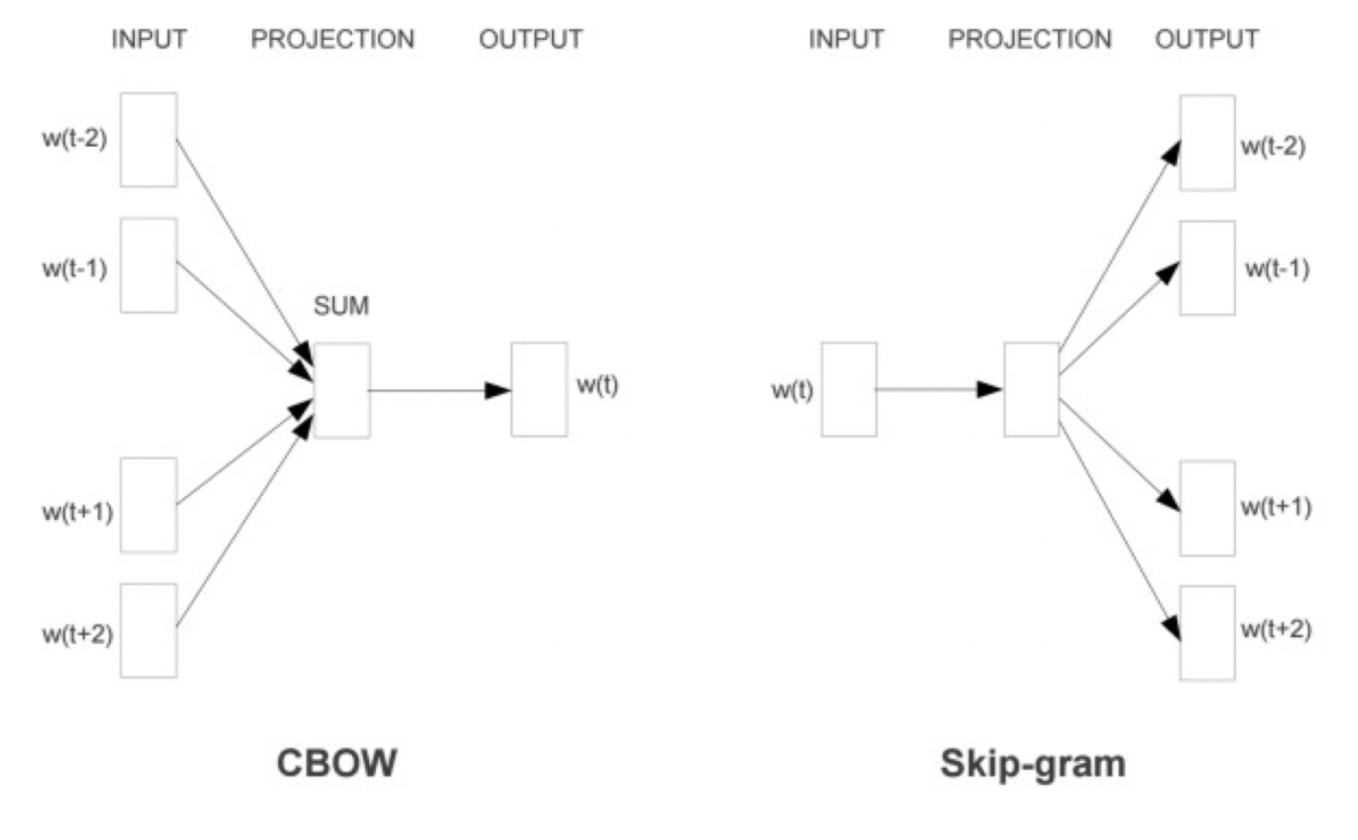I. CBOW 模型

• 即 Continuous Bags-of-Words
• 基本思想：同时考虑历史与未来，选择一个上下文窗口 C t = { w t − k , . . . , w t − 1 , w t + 1 , . . . , w t + k } \mathcal{C_t}=\{w_{t-k},...,w_{t-1},w_{t+1},..., w_{t+k}\} ，如图示为大小为 5 的窗口
• 输入层：词汇的独热向量 e w i = [ 0 ; . . . ; 1 ; . . . 0 ] ∈ [ [ 0 , 1 ] ] ∣ V ∣ e_{w_i}=[0;...;1;...0]\in [[0, 1]]^{\mathbb{|V|}}
• 词向量层：参数为 E ∈ R d × ∣ V ∣ \pmb{E}\in\mathbb{R}^{d\times|\mathbb{V}|}
• 隐含层：仅做平均操作 v C t = 1 ∣ C t ∣ ∑ w ∈ C t v w v_{\mathcal{C_t}=\frac{1}{\mathcal{|C_t|}}\sum_{w\in\mathcal{C_t}}v_w}
• 输出层：参数为 E ′ ∈ R ∣ V ∣ × d \pmb{E'}\in \mathbb{R}^{\mathbb{|V|}\times d} , P ( w t ∣ C t ) = e x p ( v C t ⋅ v w t ′ ) ∑ w ′ ∈ V exp ⁡ ( v C t ⋅ v w ′ ′ ) P(w_t|\mathcal{C_t})=\frac{exp(v_{\mathcal{C_t}}·v'_{w_t})}{\sum_{w'\in \mathbb{V}}\exp(v_{\mathcal{C_t}}·v'_{w'})} , where v w i ′ v'_{w_i} E ′ \pmb{E'} 中与 w i w_i 对应的行向量
• 词向量矩阵： E , E ′ \pmb{E, E'} 都可以作为词向量矩阵，分别表示了词在作为条件上下文或目标词时的不同性质。实际常用 E \pmb{E} , 也可以两者组合起来。
• 特点：不考虑上下文中单词的位置或者顺序，因此输入是一个词袋而非序列。

II. Skip-gram 模型

• 基本思想：在 CBOW 基础上简化为 “使用 C t \mathcal{C_t} 中的每个词作为独立的上下文对目标词进行预测”， 即 P ( w t ∣ w t + j ) P(w_t|w_{t+j})

原文献是 P ( w t + j ∣ w t ) P(w_{t+j}|w_t) , 两者等价，本书采取原文献的办法

• 隐含层向量： v w t = E w t T v_{w_t}=\pmb{E_{w_t}^T}

• 输出层：参数为 E ′ ∈ R ∣ V ∣ × d \pmb{E'}\in \mathbb{R}^{\mathbb{|V|}\times d} P ( c ∣ w t ) = e x p ( v w t ⋅ v c ′ ) ∑ w ′ ∈ V e x p ( v w t ⋅ v w ′ ′ ) P(c|w_t)=\frac{exp(v_{w_t}·v'_c)}{\sum_{w'\in\mathbb{V}}exp(v_{w_t}·v_{w'}')} ， where v w i ′ v'_{w_i} E ′ \pmb{E'} 中与 w i w_i 对应的行向量

• 词向量：与 CBOW 同。

III. 参数估计与预训练任务

• θ = { E , E ′ } \pmb{\theta}=\{\pmb{E}, \pmb{E'}\}
• 采用负对数似然函数： L ( θ ) = − ∑ t = 1 T l o g P ( w t ∣ C t ) \mathcal{L(\pmb{\theta})}=-\sum_{t=1}^TlogP(w_t|\mathcal{C_t}) ; L ( θ ) = − ∑ t = 1 T ∑ − k ≤ j ≤ k , j ≠ 0 l o g P ( w t + j ∣ w t ) \mathcal{L{\pmb{(\theta})}}=-\sum_{t=1}^T\sum_{-k\le j\le k, j\neq0}logP(w_{t+j}|w_t)

IV. 负采样

• 输出层的归一化计算效率低(当词表很大的时候)

• 样本 ( ( w , c ) (w, c) ): 正样本 c = w t + j c= w_{t+j} , 对 c c 进行若干次负采样得到： w i ~ ( i = 1 , . . . , K ) \tilde{w_i}(i=1,...,K)

• 改为：给定当前词 w w 与上下文词 c c ，最大化两者共现概率；即简化为对于 ( w , c w, c ) 的二元分类问题 (共现不共现)， P ( D = 1 ∣ w , c ) = σ ( v w ⋅ v c ′ ) P(D=1|w, c)=\sigma{(v_w·v'_c)} , P ( D = 0 ∣ w , c ) = σ ( v w ⋅ v c ′ ) = 1 − P ( D = 1 ∣ w , c ) = σ ( − v w ⋅ v c ′ ) P(D=0|w, c)=\sigma{(v_w·v'_c)}=1-P(D=1|w, c)=\sigma{(-v_w·v'_c)}

• 对数似然 l o g P ( w t + j ∣ w t ) logP(w_{t+j}|w_t) 改为： l o g σ ( v w t ⋅ v w t + j ′ ) + ∑ i = 1 K l o g σ ( − v w t ⋅ v w i ~ ′ ) log\sigma{(v_{w_t}·v'_{w_{t+j}})+\sum_{i=1}^Klog\sigma{(-v_{w_t}·v'_{\tilde{w_i}})}} , where w i ~ ∼ P n ( w ) \tilde{w_i}\sim P_n(w)

• 负采样分布的选择：假设 P 1 ( w ) P_1(w) 表示从训练语料中统计得到的 Unigram 分布，可以使用 P n ( w ) ∝ P 1 ( w ) 3 4 P_n(w)\propto P_1(w)^\frac{3}{4}

• CBOW 同理

sigma 是指 sigmoid 函数

V. 模型实现

• 不是很困难，可以查阅相关代码资料，这里只记录一些 remarkable.

• 负样本采样的实现：

• 可以在构建训练数据的时候采样，优点是训练时候不需采样效率高，但是每次的负样本一样
• 所以更喜欢在训练过程中实时地进行采样：写在 collate_fn 中
class SGNSDataset(Dataset):
def __init__(self,...):
...
self.num_neg_samples = num_neg_samples
# 传入 ns_dist 时候已经计算好了
self.ns_dist = ns_dist if ns_dist else torch.ones(len(vocab))

def __len__(self):
return len(self.data)

def __get_item(self, i):
return self.data[i]

def collate_fn(self, examples):
words = torch.tensor([ex for ex in examples], dtype=torch.long)
contexts = torch.tensor([ex for ex in examples], dtype=torch.long)
batch_size, context_size = contexts.shape
neg_contexts = []
for i in range(batch_size):
# 保证负样本中不包含当前样本的 context
ns_dist = self.ns_dist.index_fill(0, contexts[i], .0)
# 进行取样，multinomial 是均匀的，反正就是一定根据 ns_dist 取样
neg_contexts.append(torch.multinomial(ns_dist, self.num_neg_samples * context_size, replacement= True))
neg_contexts = torch.stack(neg_contexts, dim=0)
return words, contexts, neg_contexts

#  这里写出 ns_dist 的计算
unigram_dist = get_unigram_distribution(corpus, len(vocab))
ns_dist = unigram_dist ** 0.75
ns_dist /= ns_dist.sum()

• 需要维护两个词向量： w w c c 各维护一个 w_embeddingc_embedding , 然后 各设置一个 forward_wforward_c， 然后将词向量矩阵和上下文向量矩阵合并作为最终的词向量矩阵，combined_embeds = model.w_embeddings.weight + model.c_embeddings.weight 之所以这么做，是因为每个词向量要包含该词作为目标词和作为上下文的两者的信息。

(三) GloVe 词向量

I. 基本思想

传统的都是基于词与局部上下文共现信息作为自监督学习信号。另外，还有一种通过矩阵分解如 SVD 的办法 (c.f. 二(一)II.分布式表示)。但是 SVD 并不具备特别良好的几何性质。因此，GloVe 基于词向量和矩阵分解(隐式)的思想。

II. 预训练任务

• 构建共现矩阵 M \pmb{M} , 但是限制在受限窗口大小内的贡献次数(即 w w c c 的距离要足够小)
• 考虑 w w c c 的距离： M w , c = ∑ i 1 d i ( w , c ) \pmb{M_{w,c}}=\sum_i{\frac{1}{d_i(w,c)}} , where i i 表示第 i i 次共现发生
• 进行回归问题求解： v w T v c ′ + b w + b c ′ = l o g M w , c v_w^Tv_c'+b_w+b_c'=log\pmb{M_{w,c}} , 其中， v w , v c ′ v_w,v_c' 分别是 w w c c 的向量表示 (也就是他们的 GloVe 词向量)， b w b_w b c ′ b_c' 分别是相应的偏置项

III. 参数估计

• θ = { E , E ′ , b , b ′ } \pmb{\theta}=\{\pmb{E,E',b, b'}\}
• L ( θ ; M ) = ∑ ( w , c ) ∈ D f ( M w , c ) ( v w T v c ′ + b w + b c ′ − l o g M w , c ) 2 \mathcal{L}(\pmb{\theta};\pmb{M})=\sum_{(w, c)\in\mathbb{D}}f(\pmb{M_{w,c}})(v_w^Tv_c'+b_w+b_c'-log\pmb{M_{w,c}})^2
• f ( M w , c ) f(\pmb{M_{w,c}}) 表示每一个样本的权重，与共现次数有关。 f ( M w , c ) = { ( M w , c m m a x ) α i f   M w , c ≤ m m a x 1     e l s e f(\pmb{M_{w,c}})=\begin{cases}(\frac{\pmb{M_{w,c}}}{m^{max}})^\alpha & if\ \pmb{M_{w,c}}\le m^{max}\\ 1\ \ \ else\end{cases}

IV. 模型实现

和前述大同小异，除了设置了偏置项

class GloveModel(nn.Module):
def __init__(self, ...):
...
self.w_biases = nn.Embedding(vocab_size, 1)
self.c_biases = nn.Embedding(vocab_size, 1)
...
def forward_w(self, words):
w_embeds = self.w_embeddings(words)
w_biases = self.w_biases(words)
return w_embeds, w_biases

def forward_c(self, contexts):
c_embeds = self.c_embeddings(contexts)
c_biases = self.c_biases(contexts)
return c_embeds, c_biases

同样地，最后的每个单词的向量表示，也是两个嵌入的加和

combined_embeds = model.w_embeddings.weight + model.c_embeddings.weight
save_pretrained(vocab, combined_embeds.data, 'glove.vec')

(四) 评价与应用

I. 内部任务评价法

• 根据其对词义相关性或者类比推理性的表达能力进行评价
1. 词义相关性 s i m ( w a , w b ) = c o s ( v w a , v w b ) = v w a ⋅ v w b ∣ ∣ v w a ∣ ∣ ⋅ ∣ ∣ v w b ∣ ∣ sim(w_a, w_b)=cos(v_{w_a}, v_{w_b})=\frac{v_{w_a}·v_{w_b}}{||v_{w_a}||·||v_{w_b}||}

这样，就可以自己设计一个基于 KNN 的近义词检索器啦！

还可以利用含有词义相关性人工标注的数据集作为标准：WordSim353

​ 该数据集对每个单词有一个人工标准的相似度系数

​ 可以用词向量计算得到的相似度与该人工标注的相似度计算相关系数

​ 如 Spearman 相关系数或者 Pearson 相关系数

2. 类比性：对于语法或者语义关系相同的两个词对 ( w a , w b ) , ( w c , w d ) (w_a, w_b), (w_c, w_d) , 词向量在一定程度上满足 v w b − v w a ≈ v w d − v w c v_{w_b}-v_{w_a}\approx v_{w_d}-v_{w_c} 的几何性质

利用这个可以进行词与词之间的关系推理，回答诸如 “a 之于 b 相当于 c 之于 ?”等问题

w d = a r g m i n w ( c o s ( v w , v w c + v w b − v w a ) ) w_d=argmin_w({cos(v_w, v_{w_c}+v_{w_b}-v_{w_a})})

这些指标和训练数据的来源规模以及词向量维度等都有关系

1. 应用：

# initialization
self.embedding.weight.data.uniform_(-0.1, 0.1)
# copy glove vector to embedding
self.embedding.weight[idx].data.copy_(vectors[idx])

一般地，下游任务的数据集和预训练词向量用的数据集有所不同，因此，只 copy_预训练词表中存在的词，而其他不存在的词仍然使用一开始 uniform_随机初始化的词向量，并在后续训练过程中精调。当然，也可以把其他不存在的词都使用 <unk>代替 (虽然效果可能不太好)。

II. 外部任务评价法

• 根据下游任务的性能指标判断

(五) 总结六、动态词向量预训练模型

(一) 从静态到动态

• 静态词向量都基于分布式语义假设，本质是将一个词在整个语料库中的共现上下文信息聚合到该词的向量表示中，因此，一旦训练完成，词向量的表示是恒定的，不随上下文的变化而变化。然而，一词多义告诉我们，上下文不同，词义也不同。

• 因此提出上下文相关的词向量 (Contextualized Word Embedding) ，也称为动态词向量。

• 动态词向量仍然严格满足分布式语义假设。

• 循环神经网络每个隐含层的表示正好可以作为该时刻词在当前上下文条件下的向量表示，即动态词向量。

• 因此提出了 TagLM (序列标注模型)、ELMo (深度上下文相关词向量)、CoVe (双语平行语料)

• 称为：基于语言模型的动态词向量预训练

(二) ELMo 词向量

I. 双向语言模型

1. 输入表示层：• 基于字符组合的神经网络表示，以减小 OOV 的影响

即词 token 用字符 t, o, k, e, n 来表示，没什么深奥的

v c i = E c h a r e c i v_{c_i}=\pmb{E}^{char}e_{c_i} where E c h a r ∈ R d c h a r × ∣ V c h a r ∣ \pmb{E}^{char}\in \mathbb{R}^{d^{char}\times|\mathbb{V}^{char}|} , where V c h a r \mathbb{V}^{char} 为所有字符集合， d c h a r d^{char} 为字符向量维度

w t = c 1 c 2 . . . c l ‾ w_t = \overline{c_1c_2...c_l}

• 进行一维卷积对字符级向量表示序列进行语义组合 (Semantic Composition), d c h a r d^{char} 作为输入通道个数记为 N i n N^{in} , 输出向量维度作为输出通道个数 N o u t N^{out} . 可以使用不同大小的卷积核获取不同粒度的字符级上下文信息。隐含层向量的维度由每个卷积核的输出通道维数决定 (拼接而不是加和)。

• 然后，对隐含层所有位置的输出向量进行池化操作，就可以得到词 w t w_t 的定长向量表示 f t f_t .

例如，宽度分别为 {1, 2, 3, 4, 5, 6, 7}的 7 个一维卷积核，7 个 卷积核分别产生了 {7, 6, 5, 4, 3, 2, 1} 个输出向量，每个向量的维度 (输出通道数量) 分别为 { 32, 32, 64, 128, 256, 512, 1024}, 对所有位置进行池化操作, 针对每个卷积核 的池化宽度分别为 {pool7, pool6, pool5, pool4, pool3, pool2, pool1}，这样，每个卷积核的输出经过池化后分别转为一个向量，但是这 7 个向量维度不一，我们直接拼接： sum{ 32, 32, 64, 128, 256, 512, 1024}=2048, 即 f t f_t 的维度。这个 f t f_t 即初步表示了词 w t w_t

• 最后经过单层 Highway 神经网络， 得到词 w t w_t 的输入向量表示 (也可以多层)
• x t = g ⊙ f t + ( 1 − g ) ⊙ R E L U ( W f t + b ) x_t=g\odot f_t+(1-g)\odot RELU(Wf_t+b)
• 门控向量 g = σ ( W g f t + b g ) g=\sigma{(W^gf_t+b^g)}
• highway 神经网络的输出实际上是输入层 f t f_t 与隐含层 R E L U ( W f t + b ) RELU(Wf_t+b) 的线性插值结果。

这个输入层模型不是唯一的，也可以用字符级双向LSTM网络编码单词内字符串序列，欢迎尝试其他的结构。

2. 前向语言模型：以多层堆叠的 LSTM 为例，也可以使用 Transformer

P ( w 1 w 2 . . . w n ) = ∏ t = 1 n P ( w t ∣ x 1 : t − 1 ; θ l s t m → , θ s o f t m a x ) P(w_1w_2...w_n)=\prod_{t=1}^{n}P(w_t|x_{1:t-1};\overrightarrow{\theta^{lstm}},\theta^{softmax})

3. 后向语言模型：以多层堆叠的 LSTM 为例，也可以使用 Transformer

P ( w 1 w 2 . . . w n ) = ∏ t = 1 n P ( w t ∣ x t + 1 : n ; θ l s t m ← , θ s o f t m a x ) P(w_1w_2...w_n)=\prod_{t=1}^{n}P(w_t|x_{t+1:n};\overleftarrow{\theta^{lstm}},\theta^{softmax})

前后向语言模型共享了输出层 softmax 参数

通过最大化前向、后向语言模型的似然函数，就可以完成 ELMo 的预训练

II. ELMo 词向量1. 模型的编码部分(含输入表示层与多层堆叠 LSTM) (一般用 LSTM 最后一层隐含层) 便可以作为动态词向量表示。

2. 然而，不同层次的隐含层向量蕴含了不同层次或粒度的文本信息。

• 顶层编码了更多的语义信息
• 底层编码了更多的词法、句法信息
3. 最终的 ELMo 词向量：因此，ELMo 对不同层次隐含层采取了加权平均，为不同的下游任务提供了更多的组合自由度。

• 设状态向量集合: R t = { x t , h t , j ∣ j = 1 , . . . , L } \mathbb{R_t}=\{x_t,h_{t,j}|j=1,...,L\} ( L L 为层数)，其中， h t , j = [ h t , j ← ; h t , j → ] h_{t,j}=[\overleftarrow{h_{t,j}};\overrightarrow{h_{t,j}}]
• E L M o t = f ( R t , Ψ ) = γ t a s k ∑ j = 0 L s j t a s k h t , j ELMo_t=f(\mathbb{R_t, \Psi})=\gamma^{task}\sum_{j=0}^Ls_j^{task}h_{t,j}
• h t , 0 = x t h_{t,0}=x_t
• Ψ = { s t a s k , γ t a s k } \Psi=\{s^{task},\gamma^{task}\} 额外参数，前者表示每个向量的权重，可以由一组参数通过 softmax 函数归一化计算得到，一般在下游任务的训练过程中学习；后者同样和下游任务相关，当 ELMo 向量与其他向量共同作用时候，适当地缩放 ELMo 词向量

一般，ELMo 与下游任务一起训练，当然，也可以冻结词向量，不参与训练更新

III. ELMo 词向量的特点

1. 动态：上下文相关
2. 健壮：字符级输入，对于 OOV 具有健壮性
3. 层次：较大的使用自由度 (c.f. 六 (二) II.ELMo 词向量)

IV. 模型实现

• 可以看这位同学的系列代码详解笔记：ELMo代码详解, 这里记录一些关键点

• 前后向 LSTM 的输入输出(ground truth)

• 输入均为：<bos>w1w2w3...wn<eos>
• 后向输出: <pas><bos>w1w2w3...wn

• 字符级和单词级的词表等都要建立

• 字符集的保留标记新增：<BOW_TOKEN> 词首和<EOW_TOKEN> 词尾

• 我们分别计算前向语言模型和后向语言模型的输出，即分别提取两者最后一层的输出，分别计算两者的损失函数记为前向损失和后向损失，最终的损失是两个损失的平均

• 可以把输入层表示编码和双向 LSTM 编码分别给出来

• 通过公式不难发现，前后向 LSTM 的输出是独立输出的，一般将两者做拼接 (concatenation) 后作为词向量，当然，也可以灵活的进行处理，如加和等

之所以前向和后向要各自独立地进行训练与处理，是因为防止信息泄露

c.f. 七 (三) 第一条

• ELMo 可以用于模型的不同层，用于不同层的 ELMO 可以有不同的 s t a s k s^{task} , c.f. 六 (二) V.应用与评价第一条

• 也可以使用开源的 ELMo 预训练模型

• AllenNLP ：欢迎参考我的开源代码笔记本 NoahKit@ZenMoore

from allennlp.modules.elmo import Elmo, batch_to_ids
options_file = 'url-to-options.json'
weights_file = 'url-to-weights.json'
elmo = Elmo(options_file, weights_file, num_output_representations=1, dropout=0)  # num_output_representations 用于控制输出的 ELMo 向量的数目，即不同组合方式的数目，参见 六 (二) V.应用与评价第一条 以及 六 (二) VI.模型实现第七条

sentences = [['i', 'love', 'elmo'], ['hello', 'elmo']]
character_ids = batch_to_ids(sentences)
embeddings = elmo(character_ids)

# embeddings 是这个样子的
{'elmo_representations':
tensor(...),  # size=(bsz, max_length, dim)
tensor([[True, True, True],
[True, True, False]])}  # 输入文本补齐后对应的掩码矩阵

• HIT-SCIR 发布的多语言 ELMo 预训练模型

V. 应用与评价

• ELMo 非常灵活，既可以当作可训练的，也可以当作特征即插即用；既可以与静态词向量一起在模型的底层作为模型输入 [ x k ; E L M o k ] [x_k; ELMo_k] , 也可以与模型顶层或者接近输出层的隐含层相结合作为分类器 (softmax 层)的输入 [ h k ; E L M o k ] [h_k;ELMo_k]

• 回到开始，动态词向量很好地弥补了静态词向量对于一词多义现象的表达能力的不足。

七、预训练语言模型

(一) 概述

• 大数据：数据数量和质量之间进行权衡
• 大算力：NVIDIA GPU+CUDA (如 Volta V100 等), TPU (如 v2, v3 等)
• 大模型：较高的并行程度，捕获并构建上下文信息

(二) GPT

• 生成式预训练 + 判别式任务精调
• 基于单向多层 Transformer

I. 无监督预训练

• 训练任务：最大似然估计 L P T ( x ) = ∑ i l o g P ( x i ∣ x i − k . . . x i − 1 ; θ ) \mathcal{L}^{PT}(x)=\sum_ilogP(x_i|x_{i-k}...x_{i-1};\theta) , where k k 是窗口大小

其中， h [ 0 ] = e x ′ W e + W p h^{}=e_{x'}W^e+W^p h [ l ] = T r a n s f o r m e r − B l o c k ( h [ l − 1 ] ) h^{[l]}=Transformer-Block(h^{[l-1]}) P ( x ) = S o f t m a x ( h [ L ] W e T ) P(x)=Softmax(h^{[L]}W^{eT})

其中， e x ′ ∈ R k × ∣ V ∣ e_{x'}\in \mathbb{R}^{k\times\mathbb{|V|}} 为词 x ′ x' 的独热向量， W e ∈ R ∣ V ∣ × d W^e\in \mathbb{R}^{|\mathbb{V}|\times d} 为词向量矩阵， W p ∈ R n × d W^p \in \mathbb{R}^{n\times d} 为位置矩阵，这里截取其窗口的部分； L L 表示 Transformer 总层数

II. 有监督下游任务精调

• 精调的目的是在通用语义的基础上，根据下游任务的特性进行领域适配

• 例如带标签的任务： L F T ( C ) = ∑ ( x , y ) l o g P ( y ∣ x 1 . . . x n ) \mathcal{L}^{FT}(\mathcal{C})=\sum_{(x,y)}logP(y|x_1...x_n) , P ( y ∣ x 1 . . . x n ) = S o f t m a x ( h n [ L ] W y ) P(y|x_1...x_n)=Softmax(h_n^{[L]}W^y)

其中， y y 是标签， W y ∈ R d × k W^y\in\mathbb{R}^{d\times k} , k k 是标签数， C \mathcal{C} 是标注数据集

• 为了防止灾难性遗忘问题，可以在精调损失中加入一定权重的预训练损失，通常预训练损失权重系数为 0.5

III. 下游任务适配

这里主要说的是输入输出形式的适配(三) BERT

最重要模型，没有之一，建议直接看论文：BERT: Pre-training of Deep Bidirectional Transformers for Language Understanding，任何博客都不可能比原文更加详细！

• GPT 采用自回归 (ARLM, Auto-Regressive Language Model)：即根据序列历史计算当前时刻词的条件概率 l o g P ( x ) = ∑ i = 1 N l o g P ( x i ∣ x 1 : i − 1 ) logP(x)=\sum_{i=1}^{N}logP(x_i|x_{1:i-1}) , where x x 表示整个序列

如果同时使用历史和未来，会产生信息泄露！即根据历史预测当前时刻词的时候，已经知道未来啦！所以 ELMo 选择了对前向和后向分别独立地进行训练。

传统基于 N-gram 的语言模型也是自回归的

• BERT 采用自编码 (AELM, Auto-Encoding Language Model) : 类似于 MLM 这种预训练任务叫做基于自编码的预训练任务，即通过上下文重构被掩码的单词 l o g P ( x ∣ x ^ ) = ∑ i = 1 N m i l o g P ( x i ∣ x ^ ) logP(x|\hat{x})=\sum_{i=1}^Nm_ilogP(x_i|\hat{x}) , where x ^ \hat{x} 表示被掩码的序列， x x 表示整个完整序列， m i m_i 表示第 i i 个单词是否被掩码

• MLM : 掩码语言模型，是为了真正同时地依赖于历史和未来。

k k 为一个句子的掩码词数量，我们拼接它们的语义表示 (最后一层 Transformer 输出)

h m ∈ R k × d h^m\in \mathbb{R}^{k\times d} , 一般，输入层维度 e e 和隐含层维度 d d 相等，因此，直接使用 Token Embedding 矩阵即可： P i = S o f t m a x ( h i m W t T + b o u t p u t ) , i = 1 , 2 , . . . , k P_i=Softmax(h^m_iW^{tT}+b^{output}), i=1,2,...,k ，然后计算交叉熵损失。

• NSP：下一句预测

只取 h 0 h_0 , $P=Softmax{(h_0W{output})+b{output}}\ where\ W{output}\in\mathbb{R}{d\times2}$ , 然后使用交叉熵进行损失计算。

• BERT 的输入：将原始独热向量经过线性变换投影成 d i m = e dim=e

• Token Embedding : v t = e t W t , W t ∈ R ∣ V ∣ × e v^t=e^tW^t, W^t\in \mathbb{R}^{\mathbb{|V|}\times e}
• Segment Embedding : v s = e s W s , W s ∈ R ∣ S ∣ × e v^s=e^sW^s, W^s\in \mathbb{R}^{\mathbb{|S|}\times e}
• Positional Embedding : v p = e p W p , W p ∈ R N × e v^p=e^pW^p, W^p\in \mathbb{R}^{N\times e}

然后三个向量维度相同，直接相加，就是嵌入层的向量

• BERT 的 token 不是整词，而是由 WordPiece 计算出来的子词！

中文：子就是子词，词就是整词

(四) 更多掩码策略

除了上述 MLM 和 NSP 两个基本预训练任务外：再介绍三种掩码策略作为新的预训练任务

I. 整词掩码 WWM

在原来子词的基础上做掩码，即掩码掉一个整词的全部子词

II. N-gram 掩码 NM

进一步挖掘模型对连续空缺文本的还原能力

• 可以选择 短语表抽取：从语料库中抽取高频短语，但是语料库也太大了啊喂！
• 于是采用下面的办法：
2. 如果会，进一步判断 N-gram 的掩码概率 (假设最大短语长度为 4)：从 unigram 到 4-gram 概率依次减小 (40%, …, 10%), 也就是说要以概率确定一个 N
3. 对该标记及其之后 N-1 个标记进行掩码，不足 N-1 时候，以词边界进行截断
4. 掩码完毕后，跳过 N-gram，对下一个候选标记进行同样的掩码判断

由于输入仍然是子词 token, 因此不同掩码策略预训练出来的 BERT 对于下游任务是可以无缝替换的，且无须改变任何下游任务的精调代码！掩码策略只影响预训练，掩码策略对于下游任务是透明的。

(五) 预训练模型应用

I. 概述

1. 使用特征提取范式：BERT 作为特征提取输出语义表示，不进行梯度学习，后续接下游任务模型

参数少，但是对下游任务模型的设计提出了高的要求

2. 使用精调范式：BERT 也跟着训练

效果显著好，但是参数太多 (被大算力相对解决)

II. 单句文本分类 SSC

如情感分类任务 SST-2, 代码基于 hugging face

参见本人代码集 NoahAI@ZenMoore

III. 句对文本分类 SPC

如英文文本蕴含数据集 RTE

参见本人代码集 NoahAI@ZenMoore

• <cls>question<seq>passage<seq> 将问题放在前面，防止超长截断，同时，对于篇章，可以采取篇章切片的方式进行多次预测，并综合相应的答题结果得到最终的输出。
• BERT 末尾加一层全连接，用 P s = S o f t m a x ( h W s + b s ) P^s=Softmax(hW^s+b^s) P e = S o f t m a x ( h W e + b e ) P^e=Softmax(hW^e+b^e) 计算出 span 的起始和终止位置概率。将起始位置和终止位置分别计算交叉熵损失然后求平均作为总损失
• 解码方法：根据上面的概率，起始和终止分别记录 Top-K 个 (位置，概率)二元组，然后起始终止组合，每对儿概率直接乘积就好，得到 (起始位置，终止位置，概率) 的 K x K 个三元组，保证起始位置小于等于终止位置，筛选出概率最高的那个

参见本人代码集 NoahAI@ZenMoore

V. 命名实体识别 NER

如命名实体识别数据集 CoNLL-2003 NER.

• BIO 标注模式: Begin, Intermediate, Other

如 John Smith has never been to Harbin

标 B-PER, I-PER, O, O, O, O, B-LOC

• BIOES 标注模式: Begin, Intermediate, Other, End, Single

• P t = S o f t m a x ( h t W o + b o ) P_t=Softmax{(h_tW^o+b^o)} , W o ∈ R d × K W^o\in \mathbb{R}^{d\times K} , K K 表示标注模式的类别, 然后通过交叉熵损失进行学习

• 也可以在概率输出之上增加条件随机场 CRF 这一个传统命名实体识别模型

参见本人代码集 NoahAI@ZenMoore

(六) 深入理解 BERT

I. 可解释性概述

• 关于可解释性 : 分为自解释(self-explainable)事后解释(post-hoc explanation)

自解释：模型设计的可解释性

事后解释：模型行为的可解释性

目前对 BERT 的解释主要集中在 Post-hoc explanation

• 关于事后解释：需要建立模型行为与人类概念系统之间的映射

对 NLP 来说，人类概念系统即语言学特征

1. BERT 能够表达哪些语言学特征？
2. BERT 每一层的多头自注意力分别捕获了哪些关系特征？
3. 它的每一层表示是否和 ELMo 一样具有层次性？

II. 定性：自注意力可视化分析• 自注意力的本质事实上是对词(或标记)与词之间关系特征 (relational feature) 的刻画。不同类型的关系可以表达丰富的语义，比如依存关系、指代关系等。
• 挺有意思的，建议看原文献：What Does BERT Look At? An Analysis of BERT’s Attention

III. 定量：探针实验

1. 核心思想：设计特定的探针，对于待分析对象(如自注意力或隐含层表示)进行特定行为分析。

2. 探针：通常是非参或非常轻量的参数模型(如线性分类器)，它接受待分析对象作为输入，并对特定行为进行预测，而预测的准确度可以作为待分析对象是否具有该行为的衡量指标3. 也可以对预训练编码器的隐含层表示直接进行探针实验，这里的探针可以是一个简单的线性分类器，利用隐含层表示作为特征在目标任务上进行训练，从而根据该任务的表现对预训练模型隐含层表示中蕴含的语言学特征进行评估4. 对于更加复杂的结构预测类任务，如句法分析等，也可以设计针对性的结构化探针

八、预训练语言模型进阶

• 模型优化：如何进一步改进现有的预训练模型？
• 长文本处理：如何更好地建模长文本？
• 蒸馏与压缩：如何提升预训练语言模型的效率？
• 生成式模型：如何设计出更有效的生成式预训练语言模型？

(一) 模型优化

• 自编码语言模型不具备自回归的预测性
• 自回归语言模型单向性
• BERT : 太重，改进空间大

I. XLNet

• 基本特点：

1. 使用自回归语言模型结构，使得每个单词的预测存在依赖性，同时避免了“预训练-精调”不一致问题。(这也是 BERT 的缺点，GPT 的优点)
2. 引入了自编码语言模型中的双向上下文，能够利用好更加丰富的上下文信息，而不像传统自回归模型那样只有单向信息。(这也是 BERT 的优点，GPT 的缺点)
3. 使用了 Transformer-XL 作为主体框架，比 Transformer 拥有更好的性能。
• Transformer-XL 在 “八 (二) 长文本处理” 中介绍。

• 输入层表示：采用和 BERT 一样的三种 Embedding 相加的方式, 记为 v x i v_{x_i}

• 排列语言模型 (Permutation Language Model)

• 对句子建模顺序进行了更改, 如 P ( x ) = P ( x 3 ) P ( x 2 ∣ x 3 ) P ( x 4 ∣ x 3 x 2 ) P ( x 1 ∣ x 3 x 2 x 4 ) P(x)=P(x_3)P(x_2|x_3)P(x_4|x_3x_2)P(x_1|x_3x_2x_4)

原顺序为：1 -> 2 -> 3 -> 4

改成了 ：3 -> 2 -> 4 -> 1

不难证明两种顺序的 P ( x ) P(x) 是相等的

• 最大化对数似然函数： E z ∼ Z N [ l o g P ( x ∣ z ) ] = E z ∼ Z N [ ∑ i = 1 N P ( x z i ∣ x z 1 : i − 1 , z i ) ] \mathbb{E_{z\sim{Z_\mathit{N}}}}[logP(x|z)]=\mathbb{E_{z\sim{Z_\mathit{N}}}}[\sum_{i=1}^NP(x_{z_i}|x_{z_{1:i-1}},z_i)]

Z N \mathbb{Z}_N 表示所有可能的排列方式

z i z_i 表示新顺序

• 在下游任务阶段： P ( x z i = x ∣ x z 1 : i − 1 ) = e x p ( v x T h x z 1 : i − 1 ) ∑ x ′ e x p ( v x ′ T h x z 1 : i − 1 ) P(x_{z_i}=x|x_{z_{1:i-1}})=\frac{exp(v_x^Th_{x_{z_{1:i-1}}})}{\sum_{x'}exp(v_{x'}^Th_{x_{z_{1:i-1}}})}

• 在预训练阶段： P ( x z i = x ∣ x z 1 : i − 1 ) = e x p ( v x T g ( x z 1 : i − 1 , z i ) ) ∑ x ′ e x p ( v x ′ T g ( x z 1 : i − 1 , z i ) ) P(x_{z_i}=x|x_{z_{1:i-1}})=\frac{exp(v_x^Tg(x_{z_{1:i-1}}, z_i))}{\sum_{x'}exp(v_{x'}^Tg(x_{z_{1:i-1}}, z_i))} , where g ( ⋅ ) g(·) 表示一种依赖于目标位置 z i z_i 的隐含层表示方法，详见下面的“双流自注意力机制"

之所以不用下游任务阶段的那个式子，是因为：

h x z 1 : i − 1 h_{x_{z_{1:i-1}}} 是不依赖于目标位置 z i z_i 的，也就是说，对于不同的目标位置，这个式子总会产生一样的概率分布，这将无法满足最大化对数似然函数的建模要求

• 双流自注意力机制 (Two-stream Self-attention)

• g ( ⋅ ) g(·) 应该满足两个要求：

1. 预测 x z i x_{z_i} 时候，只能知道位置信息 z i z_i 不能知道单词信息 x z i x_{z_i}
2. 预测 x z j   ( j > i ) x_{z_j}\ (j>i) 的时候，又需要编码 x z i x_{z_i} 以提供完整的上下文信息
• 因此，改变 Vanilla Transformer ，同一个单词具有两种表示方法：

1. 内容表示 h z i h_{z_i} : Vanilla Transformer 的表示，可以同时建模 x z i x_{z_i} 及其上下文
2. 查询表示 g z i g_{z_i} : 能建模上下文信息 x z 1 : i − 1 x_{z_{1:i-1}} 以及目标位置 z i z_i , 但不能知道 x z i x_{z_i}
• 内容表示： h z i [ 0 ] = v x i h_{z_i}^{}=v_{x_i} , then h z i [ l ] ← T r a n s f o r m e r − B l o c k ( Q = h z i [ l − 1 ] , K = h z ≤ i [ l − 1 ] , V = h z ≤ i [ l − 1 ] ; θ ) h_{z_i}^{[l]}\leftarrow Transformer-Block(Q=h_{z_i}^{[l-1]}, K=h_{z_{\le i}}^{[l-1]}, V=h_{z_{\le i}}^{[l-1]};\theta)

• 查询表示： g z i [ 0 ] = w g_{z_i}^{}=w , where w w 是随机初始化的可训练向量，then

g z i [ l ] ← T r a n s f o r m e r − B l o c k ( Q = g z i [ l − 1 ] , K = h z 1 : i − 1 [ l − 1 ] , V = h z 1 : i − 1 [ l − 1 ] ; θ ) g_{z_i}^{[l]}\leftarrow Transformer-Block(Q=g_{z_i}^{[l-1]}, K=h_{z_{1:i-1}}^{[l-1]}, V=h_{z_{1:i-1}}^{[l-1]};\theta)

• 主要通过改变注意力掩码矩阵实现，分别记为 M i , j h M_{i,j}^h M i , j g M_{i,j}^g

表示 第 i 个词和第 j 个词之间有无关联

g , h g,h 分别表示查询表示和内容表示• 使用最后一层的查询表示计算预训练阶段概率分布

• 部分预测 (Partial Prediction)

• 为了收敛速度：排列是随机的而且很多
• 因此只截取末尾 1 K \frac{1}{K} 的子序列进行预测，即先随机排列，然后选择子序列，分为 non-target 和 target
• non-target : 不计算查询流，利用下游任务阶段的那个式子，使用 Vanilla Transformer
• target : 使用上述的完整方法进行预测
• 相对块编码 (Relative Segment Encodings)

• 提升了模型对不同输入形式的泛化能力，甚至可以多于两个输入的 Segment.
•这里记录一个问题及其解答：II. RoBERTa

并没有大刀阔斧的改进 BERT，而只是针对 BERT 的每一个设计细节进行了详尽的实验找到了 BERT 的改进空间。

1. 动态掩码：原始方式是构建数据集的时候设置好掩码并固定，改进方式是每轮训练将数据输入模型的时候才进行随机掩码

2. 舍弃 NSP 任务

• 四种：文本对输入+NSP, 句子对输入+NSP，跨文档整句输入，文档内整句输入 (后两个不用 NSP)
• 实验表明：除了情感分类任务 SST-2, 其他任务不使用 NSP 均能提升性能
• 文档内整句输入 比 跨文档整句输入 性能好，但是前者导致批次大小是个可变量，因此，最终选择了跨文档整句输入并舍弃了 NSP 任务
3. 更多训练数据，更大批次，更长的预训练步数

4. 更大的词表：使用 SentencePiece 这种字节级别的 BPE 词表而不是 WordPiece 字符级别的 BPE 词表，这样，不会出现未登录词的情况

比如使用英文的 BERT 词表，也许输入德文会不出现 UNK ，但是输入日文和中文，会有很多 UNK，因为中文的子词不在英文的 BERT 词表中

但是使用 RoBERTa 词表，因为是字节级别，即便是中日文输入，也不会出现哪怕一个 UNK

III. ALBERT

BERT 参数量相对较大，因此提出 A Lite BERT.

• 词向量因式分解

• 问题一：BERT 的词向量维度和隐含层维度相同，根据 embedding 的式子不难看出，这个词向量是上下文无关的 (因为每个单词独立的通过线性变换投影到了词向量空间上) ，而 BERT 的 Transformer 层可以学到充分的上下文信息因此隐含层向量维度 H H 应该远远大于词向量维度 E E

• 问题二：当增大 H H 提升模型性能时，由于 E E 恒等变大，导致词向量矩阵参数量激增，而词向量的信息就那么多，因此词向量矩阵更新是稀疏的，参数利用率不高

• 解决：令 H ≠ E H\neq E , 并在词向量空间之后新增全连接层将 E E 投影到 H H

原来： O ( V H ) = O ( V E ) O(VH)=O(VE)

改进后 : O ( V E + E H ) O(VE+EH)

• 跨层参数共享

••• 句子顺序预测 SOP

• 正例不变，负例对调文本对
• 学习到细微的语义差别和语篇连贯性
• NSP 任务不好是因为太简单，SOP 相对困难一些

IV. ELECTRA

• 基本思想：生成器-判别器

• 生成器：即一个 MLM + Sampling, P G ( x m ∣ x ) = S o f t m a x ( h m G W e T ) P^G(x_m|x)=Softmax(h_m^GW^{eT})

x x 是一句带 [MASK] 的文本， m m 是掩码下标

W e W^e 为词向量矩阵

采样：按照概率采样出掩码住的词汇

• 判别器：输入=采样后的句子，输出为 替换词检测 (RTD) P D ( x i s ) = σ ( h i D w ) , ∀ i ∈ M P^D(x_i^s)=\sigma(h_i^Dw), \forall i\in \mathbb{M}

x s x^s 是采样的句子， w ∈ R d w\in \mathbb{R}^d 是全连接层权重， M \mathbb{M} 是所有经过掩码的单词位置下标

RTD：判断一个词是不是原句(不带掩码的原句)对应位置的单词

• 预训练任务：

• 生成损失： L G = − ∑ i ∈ S l o g P G ( x i ) \mathcal{L}^G=-\sum_{i \in \mathbb{S}}logP^G(x_i)
• 判别损失： L D = − ∑ i ∈ S [ y i l o g P D ( x i s ) + ( 1 − y i ) l o g ( 1 − P D ( x i s ) ) ] \mathcal{L}^D=-\sum_{i\in\mathbb{S}}[y_ilogP^D(x_i^s)+(1-y_i)log(1-P^D(x_i^s))]
• 总损失： m i n θ G , θ D ∑ x ∈ X L G ( x , θ G ) + λ L D ( x , θ D ) min_{\theta^G,\theta^D}\sum_{x\in \mathcal{X}}\mathcal{L}^G(x,\theta^G)+\lambda\mathcal{L}^D(x,\theta^D)
• 采样没有梯度回传，因此两个损失
• 下游任务精调：只使用判别器，抛弃生成器

• 改进：

• 生成器和判别器分别一个 BERT，太大了：缩放生成器 BERT 参数

缩放比通常为 1/4 至 1/2

• 词向量因式分解

• 两器参数共享：只能在输入层进行参数共享，包括词向量矩阵和位置向量矩阵。

V. MacBERT

哈工大-讯飞联合实验室的作品，用于解决“预训练-精调”不一致问题

• “预训练-精调”不一致问题：就是上面说的预训练时候有掩码标记，下游没有掩码标记，人为造词，两者不一
• 与 BERT 的不同：
1. 整词掩码和 N-gram 掩码
2. 使用相似词替代掩码标记，没有相似词时，使用词表随机词
3. 使用 SOP 替换 NSP 任务
• 叫做：基于文本纠错的掩码语言模型 (MLM as correction, Mac)

VI. 总结(二) 长文本处理

• 自注意力模型复杂度 O ( N 2 ) O(N^2) , 时间和空间都是
• 传统长文本处理方法：切分输入文本为固定长度，然后将多片的处理结果进行投票或者平均或者拼接

I. Transformer-XL

• 基本思想：解决切分文本每块间的失联问题以及滑动窗口处理方式的低效性

切分文本每块间的失联问题: 如 x 分为 (x_1,…, x_4), (x_5,…x_8), 两块儿分别处理，(x_5,…x_8)看不到前面四个

滑动窗口处理方式的低效性： (x_1,…, x_4)处理一次， (x_5,…x_8)还要再处理一次

• 状态复用的块级别循环 (Segment-level Recurrence with State Reuse)

• 假设两块: s τ = x τ , 1 . . . x τ , n s_{\tau}=x_{\tau,1}...x_{\tau,n} s τ + 1 = x τ + 1 , 1 . . . x τ + 1 , n s_{\tau+1}=x_{\tau+1,1}...x_{\tau+1,n}

• 隐含状态递推计算

• h ~ τ + 1 [ l − 1 ] = [ S G ( h τ [ l − 1 ] ) ∘ h τ + 1 [ l − 1 ] ] \tilde{h}_{\tau+1}^{[l-1]}=[SG(h_\tau^{[l-1]})\circ h_{\tau+1}^{[l-1]}] , S G ( ⋅ ) SG(·) 表示停止梯度传输， ∘ \circ 表示沿着长度拼接
• q τ + 1 [ l ] = h τ + 1 [ l − 1 ] W q T q_{\tau+1}^{[l]}=h_{\tau+1}^{[l-1]}W^{qT} , k τ + 1 [ l ] = h ~ τ + 1 [ l − 1 ] W k T k_{\tau+1}^{[l]}=\tilde{h}_{\tau+1}^{[l-1]}W^{kT} , v τ + 1 [ l ] = h ~ τ + 1 [ l − 1 ] W v T v_{\tau+1}^{[l]}=\tilde{h}_{\tau+1}^{[l-1]}W^{vT}
• h τ + 1 [ l ] = T r a n s f o r m e r − B l o c k ( q τ + 1 [ l ] , k τ + 1 [ l ] , v τ + 1 [ l ] ) h_{\tau+1}^{[l]}=Transformer-Block(q_{\tau+1}^{[l]}, k_{\tau+1}^{[l]}, v_{\tau+1}^{[l]})
• 注意： h τ + 1 [ l ] h_{\tau+1}^{[l]} h τ [ l − 1 ] h_\tau^{[l-1]} 之间的循环依赖性使得存在向下一层的计算依赖，这与 RNN 的同层循环机制是不同的。因此，最大的可能依赖长度随着块的长度 n n 和层数 L L 呈线性增长，即 O ( n L ) O(nL)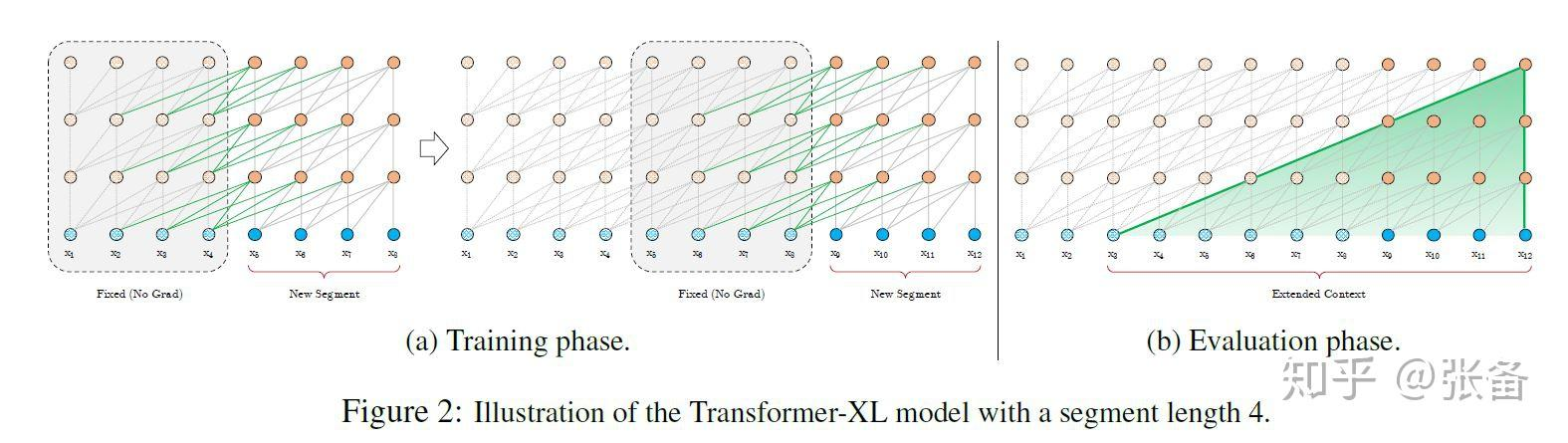这与 RNN 的 BPTT 类似，然而，在这里是将整个序列的隐含层状态全部缓存，而不是像 BPTT 那样只会保存最后一个状态。

• 这个设计还能加快测试速度，达 1800 倍以上的加速

比如两个片段：[1, 2, 3, 4] 和 [5, 6, 7, 8]

因为原来解码的时候是这样的: [1, 2, 3, 4]->5, [2, 3, 4, 5]->6,…

现在是这个样子的：[1, 2, 3, 4]->5, [1, 2, 3, 4](cache)+->6, [1, 2, 3, 4](cache)+[5, 6]->7

• 相对位置编码

• 但是，如何区分不同块的相同位置，因为每个块用的绝对位置编码是一致的

• 因为位置信息的重要性主要体现在注意力矩阵的计算上，用于构建不同词之间的关联关系

a i , j = v x i T W q T W E v x j + v x i T W q T W R R i − j + u E T W E v x j + u R T W R R i − j a_{i,j}=v_{x_i}^TW^{qT}W^Ev_{x_j}+v_{x_i}^TW^{qT}W^RR_{i-j}+u^{ET}W^Ev_{x_j}+u^{RT}W^RR_{i-j}

v v 表示词向量， W W 表示可以训练的矩阵， u ∈ R d u\in\mathbb{R}^d 表示可以训练的位置向量

R ∈ R N × d R\in \mathbb{R}^{N\times d} 表示相对位置矩阵，是一个不可训练的正弦编码矩阵，其第 i行表示相对位置间隔为 i 的位置向量

• 第一项：基于内容的相关度：计算查询 x i x_i 与键 x j x_j 的内容之间关联信息
• 第二项：内容相关的位置偏置：计算查询的内容与键的位置编码之间的关联信息
• 第三项：全局内容偏置：计算查询的位置编码与键的内容之间的关联信息
• 第四项：全局位置偏置：计算查询与键的位置编码之间的关联信息

查询 x i x_i 的内容： W q v x i W^qv_{x_i}

查询 x i x_i 的位置编码： u E u^E u R u^R

x j x_j 的内容： W E v x j W^Ev_{x_j}

x j x_j 的位置编码： W R R i − j W^RR_{i-j}

• W E W^E 不是词向量矩阵！！！

• 计算 a a b b 的关联信息： a T b a^Tb

• 然后用 a i , j a_{i,j} 这个注意力矩阵来计算 Transformer 中的 attention

II. Reformer

• 局部敏感哈希注意力

• 合并查询与键 : Q K QK 共享的 Transformer

• 局部注意力：在 Attention 计算的 Softmax 前，只取与当前查询关联度最高的 n n 个词，而不是全部词

• 局部敏感哈希 (Locality-Sensitive Hashing, LSH) : 用于解决高维空间下寻找最近邻元素的问题

• 给定一个查询向量，怎么计算与其最近邻的几个元素呢？
• 目标是设计一个哈希函数 h ( x ) h(x) ，使得向量 x x 周围的向量以较高概率具有一样的哈希值，而较远的向量具有不一样的哈希值
• 经典 LSH 方法：哈希函数定义为 h ( x ) = a r g m a x ( [ x R ; − x R ] ) h(x)=argmax([xR;-xR]) , where R ∈ R d × b / 2 R\in\mathbb{R}^{d\times b/2} 是一个随机矩阵， [ ⋅ ; ⋅ ] [·;·] 表示向量拼接操作
• 注意力计算• 多轮局部敏感哈希：哈希的过程即为信息压缩的过程，实际上会以很小的概率将相似向量放在不同的桶里，因此多做几轮取并集，这个可以显著提升准确性

• 可逆 Transformer

• 受到可逆残差网络 RRN 的启发：任意一层的激活值都可以通过后续层的激活值进行还原，因此在进行后向梯度计算时候，不需要再保存每个中间层的激活值，只需要通过可逆计算获得相应值即可。

计算耗时，但用时间换了空间。

残差网络一般表达为： Y = X + F ( X ) Y=X+\mathcal{F}(X) F , G \mathcal{F}, \mathcal{G} 是残差函数

我们分解 X , Y X, Y 为: ( X 1 , X 2 ) (X_1, X_2) ( Y 1 , Y 2 ) (Y_1, Y_2)

然后变换为： Y 1 = X 1 + F ( X 2 ) Y_1=X_1+\mathcal{F}(X_2) Y 2 = X 2 + G ( Y 1 ) Y_2=X_2+\mathcal{G}(Y_1)

这样，就可以 X 2 = Y 2 − G ( Y 1 ) X_2=Y_2-\mathcal{G}(Y_1) X 1 = Y 1 − F ( X 2 ) X_1=Y_1-\mathcal{F}(X_2) 进行逆向计算

• 将 RRN 引入 Transformer 的残差计算中, Y 1 = X 1 + A t t e n t i o n ( X 2 ) Y_1=X_1+Attention(X_2) Y 2 = X 2 + F F N ( Y 1 ) Y_2=X_2+FFN(Y_1) , 同时去掉层归一化 LN

• 可逆 Transformer 中的分块机制：进一步降低前馈神经网络的内存占用 (下图分成 c c 块)

[外链图片转存失败,源站可能有防盗链机制,建议将图片保存下来直接上传(img-lFCB1OCB-1628856051792)(https://www.zhihu.com/equation?tex=%5Cbegin%7Bequation%7D+%5Cbegin%7Bsplit%7D+y_2+%26%3D+x_2+%2B+FFN%28y_1%29+%5C%5C+%26%3D+%5By_2%5E%7B%281%29%7D%3B+y_2%5E%7B%282%29%7D%3B…%3By_2%5E%7B%28c%29%7D%5D+%5C%5C+%26%3D+%5Bx_2+%5E%7B%281%29%7D+%2B+FFN%28y_1+%5E%7B%281%29%7D%29%3B+x_2+%5E%7B%282%29%7D+%2B+FFN%28y_1+%5E%7B%282%29%7D%29%3B…%3B+x_2+%5E%7B%28c%29%7D+%2B+FFN%28y_1+%5E%7B%28c%29%7D%29%5D+%5Cend%7Bsplit%7D+%5Cend%7Bequation%7D)]

III. Longformer

基于稀疏注意力机制，将输入文本序列最大长度扩充为 4096.

• 滑动窗口注意力：每个词只与相邻 k 个词计算注意力

类似卷积，通过层的叠加可以逐步扩展感受野，如第 L 层的感受野为 Lk, 复杂度 O ( N ) O(N)• 扩张滑动窗口注意力：引入扩张率 d, 与隔 d - 1 个词计算注意力

类似扩张卷积，L 层的感受野为 Ldk, 复杂度 O ( N ) O(N)• 全局注意力：根据任务特点选择全局注意力要关注的位置

如分类任务中 [CLS] 是全局可见的，问答类任务中，问题中的所有单词是全局可见的

全局可见的词数量远小于序列长度，因此复杂度仍然是 O ( N ) O(N)IV. BigBird

• 随机注意力: 针对每个词随机选择 r 个词参与注意力运算

• 滑动窗口注意力

• 全局注意力

• 内部 Transformer 组建 (Internal Transformer Construction, ITC) 模式: 同 Longformer
• 外部 Transformer 组建 (External Transformer Construction, ETC) 模式: 在输入序列中插入额外的全局标记,使其能够看到所有词,反之亦然(对方也能看到), 而非选择.
• BigBird : 三种混合• 证明 BigBird 是序列建模函数的通用近似方法,并且是图灵完备的

V. 总结

Transformer 变体 (aka. X-former) 综述

(三) 模型蒸馏与压缩

减小参数、加快运行效率？预训练语言模型压缩技术！

目前最常用的预训练语言模型压缩技术是知识蒸馏技术.

知识蒸馏技术 (Knowledge Distillation, KD)：通常由教师模型和学生模型组成，将知识从教师模型传到学生模型，使得学生模型尽量与教师模型相近，在实际应用中，往往要求学生模型比教师模型小并基本保持原模型的效果。

I. DistilBERT

• 学生模型: 六层的 BERT, 同时去掉了标记类型向量 (Token-type Embedding, 即 Segment Embedding), 和池化模块，使用教师模型的前六层进行初始化

• 教师模型: BERT-base

• 训练：与 BERT 基本一致，只是损失函数有所区别 (只有 MLM，没有 NSP)

• 知识蒸馏方法

• 符号： s i , t i s_i, t_i 表示概率输出, y i y_i 表示标签, , h t , h s h^t, h^s 表示最后一层隐含层输出

• 有监督 MLM 损失 L s − m l m = − ∑ i y i l o g ( s i ) , \mathcal{L}^{s-mlm}=-\sum_i{y_ilog(s_i)}, 称为硬标签(ground-truth)

• 蒸馏 MLM 损失 L d − m l m = − ∑ i t i l o g ( s i ) \mathcal{L}^{d-mlm}=-\sum_i{t_ilog(s_i)} , 称为软标签(teature prob output)

DistilBERT 在计算输出概率时采用了带有温度系数的 Softmax 函数：

P i = e x p ( z i / T ) ∑ j e x p ( z j / T ) P_i=\frac{exp(z_i/T)}{\sum_jexp(z_j/T)} where z i , z j z_i, z_j are not activated.

training step : T=8

inference step: T=1

• 词向量余弦损失 : 用来对齐教师模型和学生模型的隐含层向量的方向， L c o s = c o s ( h t , h s ) \mathcal{L}^{cos}=cos(h^t, h^s)

• 总损失为三个损失的相加，比例为 1:1:1

II. TinyBERT

• 知识蒸馏方法：教师模型为 12 层的 BERT-base，学生模型为 4 层 BERT

• 词向量层蒸馏 L e m b = M S E ( v s W e , v t ) \mathcal{L^{emb}}=MSE(v^sW^e,v^t)

教师模型和学生模型的词向量维度不一定相等，因此需要投影到同一维度

• 中间层蒸馏：中间层匹配损失=隐含层蒸馏损失+注意力蒸馏损失

• 映射关系： g ( i ) = j g(i)=j , 将学生模型的第 i i 层和教师模型的第 j j 层联系起来, TinyBERT 使用的是 g ( i ) = 3 i g(i)=3i

• L h i d ( i , j ) = M S E ( h s [ i ] W h , h t [ j ] ) \mathcal{L^{hid}}(i,j)=MSE(h^{s^{[i]}}W^h,h^{t^{[j]}})

• L a t t ( i , j ) = 1 K ∑ k = 1 K M S E ( A s [ i ] , A t [ j ] ) \mathcal{L^{att}}(i,j)=\frac{1}{K}\sum_{k=1}^KMSE(A^{s^{[i]}},A^{t^{[j]}})

K 表示注意力头数， A A 是一个 n × n n\times n 的注意力矩阵

这里使用的注意力矩阵未经过 Softmax 激活

• L m i d = ∑ i , j [ L h i d ( i , j ) + L a t t ( i , j ) ] ,     s . t . g ( i ) = j \mathcal{L^{mid}}=\sum_{i,j}[\mathcal{L^{hid}(i,j)}+\mathcal{L}^{att}(i,j)],\ \ \ s.t. g(i)=j

• 预测层蒸馏：和 DistilBERT 的软标签蒸馏方法一样

对于 TinyBERT， 温度系数为 1

• 总损失： L m o d e l = ∑ m = 0 M + 1 λ m L l a y e r ( S m , T g ( m ) ) \mathcal{L}_{model}=\sum_{m=0}^{M+1}\lambda_m\mathcal{L}_{layer}(S_m, T_{g(m)}) , where m = i , M 即 学 生 模 型 层 数 m=i, M 即学生模型层数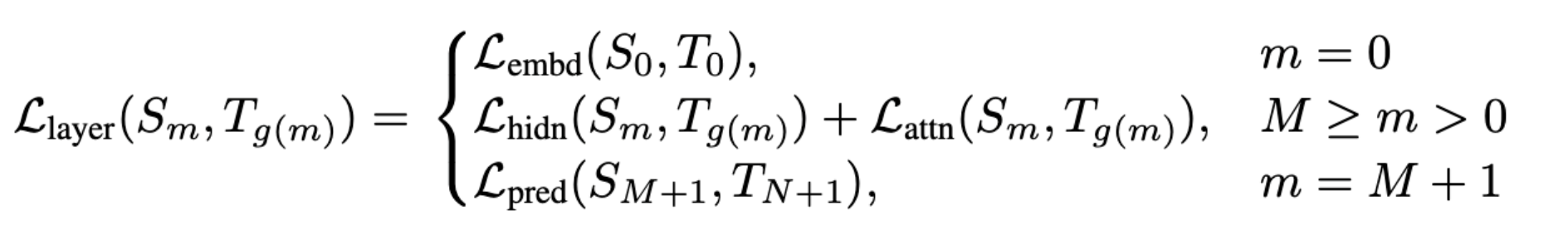• 两段式蒸馏：即在预训练和下游任务精调均进行蒸馏

•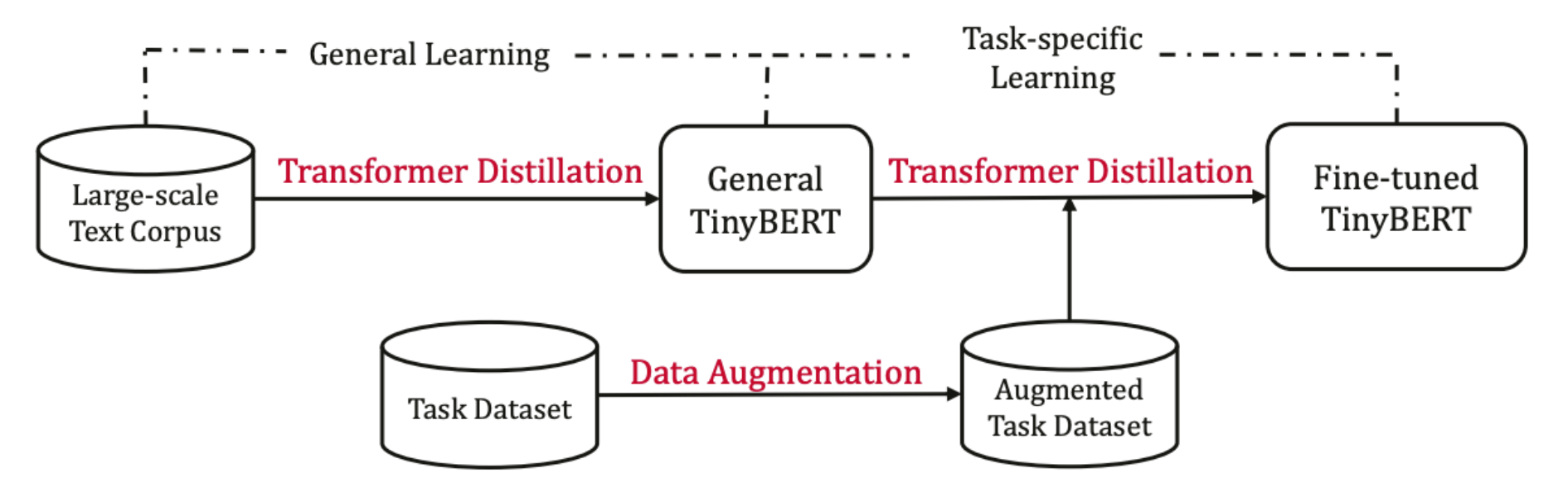• 通用蒸馏：教师模型仍使用未精调但经过预训练的 BERT-base，但是没有使用预测层蒸馏损失，因为通用蒸馏重点学习 BERT 主体部分的表示能力
• 特定任务蒸馏：使用了经过精调的 BERT-base 作为教师模型，使用数据增广后的下游任务数据进行训练
• 数据增广：将输入文本中的部分词汇通过 BERT 和 Glove 生成的词向量计算其最近似的词并进行替换

III. MobileBERT

• 学生模型和教师模型的层数是一致的，都是 12 层，因此无需映射函数

• 知识蒸馏方法： L = α L m l m + L n s p + ( 1 − α ) ( L h i d + L a t t ) \mathcal{L}=\alpha\mathcal{L}^{mlm}+\mathcal{L}^{nsp}+(1-\alpha)(\mathcal{L}^{hid}+\mathcal{L}^{att})

• 有监督 MLM 损失和有监督 NSP 损失：和原版 BERT 一致，又称预训练蒸馏

• 隐含层蒸馏损失：和 TinyBERT 一致，又称特征图迁移

• 注意力蒸馏损失：将 TinyBERT 的 MSE 换为 KL-divergence，又称注意力图迁移

KL 散度不是对称的，教师模型在前，学生模型在后

将特征图迁移和注意力图迁移称为知识提炼

• 知识迁移方案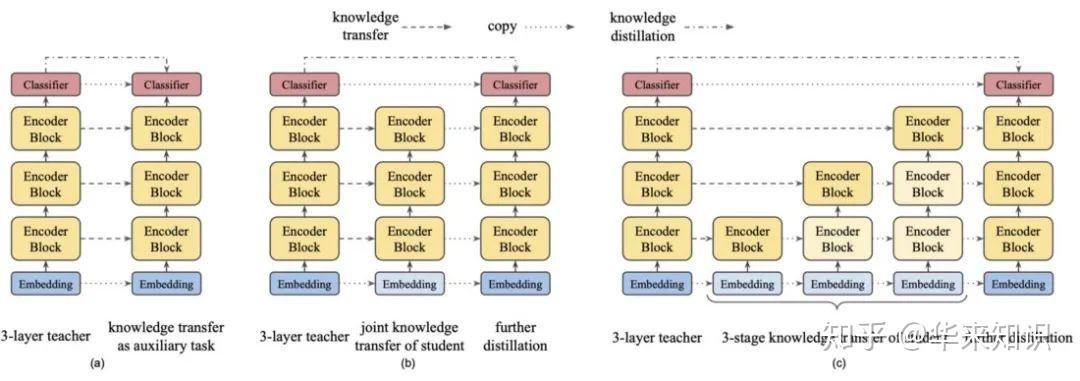蓝色：嵌入；黄色：知识提炼；红色：预训练蒸馏

深色：可训练的；浅色：参数固定而不可训练的

• Auxiliary Knowledge Transfer : 辅助知识迁移，知识提炼和预训练蒸馏同时进行
• Joint Knowledge Transfer : 联合知识迁移，先知识提炼再预训练蒸馏
• Progressive Knowledge Transfer：渐进式知识迁移，逐层进行，显著优于其他非渐进式的直接蒸馏方法

(四) 生成模型

• 条件式生成 (Conditional Generation): 条件可以是源语言(翻译任务)、文档(文本摘要任务)、属性或主题(可控文本生成任务)等
• 之前所述基本上都只是编码器 Encoder, 对于文本生成任务，需要一个强大的解码器 Decoder

I. BART

• 基本思想：去噪自编码器，仍然采用自编码器的策略 (c.f. 七 (三) BERT)，但是通过对含有噪声的输入文本去噪重构进行预训练

• 基本结构：基于 Transformer 的 Seq2Seq 结构，即编码器是双向 Transformer Encoder, 解码器是单向的自回归的 Transformer Decoder. 不过，激活函数不再使用 ReLU，而使用GeLU，参数根据正态分布 N ( 0 , 0.02 ) \mathcal{N(0, 0.02)} 进行初始化

编码器的最后一层隐含层表示会作为“记忆”参与解码器每一层的计算

这个不难理解，看一眼基于 Transformer 的 Seq2Seq 的结构图就一目了然

• 噪声引入方式：

• 单词掩码：BERT 原生 MLM 的掩码方式

不过，BERT 的 MLM 是自编码方式独立预测掩码位置的单词，而 BART 是通过自回归的方式顺序地生成

• 单词删除：和掩码不同，模型不仅要预测缺失什么单词，还要确定缺失单词的位置

• 句子排列变换：注意是句子排列变换而不是单词排列变换，因此，这个语义理解是文档级别的

• 文档旋转变换：类似于抽象代数里面的轮换，实现时，先选择一个单词，然后以该单词为首词

• 文本填充：按照参数为 3 的泊松分布采样多个片段长度，然后对各片段长度用单个掩码进行标记替换，如果片段长度为0，则只是插入一个掩码标记。这要求模型具备预测缺失文本片段长度的能力

基于文本填充任务得到的预训练模型在下游任务中表现普遍更好，在此基础上增加句子排列变换去噪任务能够带来小幅的额外提升

''' BART 文本填充能力的演示 '''
from transformers import BartTokenizer, BartForConditionalGeneration

input = 'un chief says there is <mask> in Syria'
batch = tokenizer(input, return_tensors= 'pt')
output = tokenizer.batch_decode(output_ids, skip_special_tokens= True)
print(output)  #['un chief says there is no war in Syria']

• 模型精调：不同的下游任务精调方式有所不同

• 序列分类：解码器和编码器使用相同的输入，将解码器最终时刻的隐含层状态当作输入文本的向量表示，因为解码器的最终时刻会额外添加一个类似于 [CLS] 的特殊标记，以该标记的隐含层状态当作文本表示。

• 序列标注：编码器和解码器使用相同的输入，解码器各个时刻的隐含层状态将作为该时刻单词的向量表示用于类别预测

• 文本生成：可以直接用于条件式文本生成任务，如 Abstractive QA, Abstractive Summarization

• 编码器输入：作为条件的输入文本
• 解码器：以自回归的方式生成对应的目标文本
• 机器翻译：由于源语言和目标语言使用的词汇集合不同，因此需要将编码器的输入表示层替换为一个小型的 Transformer 层(随机初始化编码器)来将源语言中的词汇映射到目标语言的输入表示空间。但是，由于除了这个源语言编码器外，其余部分都经过了预训练，两者训练步调不一致，因此：首先固定大部分参数只训练源语言编码器、BART 位置向量和预训练编码器第一层的自注意力输入投射矩阵，然后再对所有的参数进行少量迭代训练。

BART 在判别任务上的表现也很优异,甚至与 RoBERTa 持平

II. UniLM

• 基本思想：如何统一双向语言模型 BERT 和单向语言模型 Decoder of BART 呢? 这两者的区别在于：对于每一时刻隐含层表示的计算可以使用序列中的哪部分信息。因此，可以通过使用不同的自注意力掩码矩阵控制每个词的注意力范围，从而实现对于信息流的控制

• 自注意力矩阵 A l = S o f t m a x ( Q l K l T d + M ) , M i , j = { 0 , i f  i to j visible − ∞ , o t h e r w i s e A_l=Softmax(\frac{Q_lK_l^T}{\sqrt{d}}+M), M_{i,j}=\begin{cases} 0, & if\ \text{i to j visible}\\ -\infty, & otherwise \end{cases}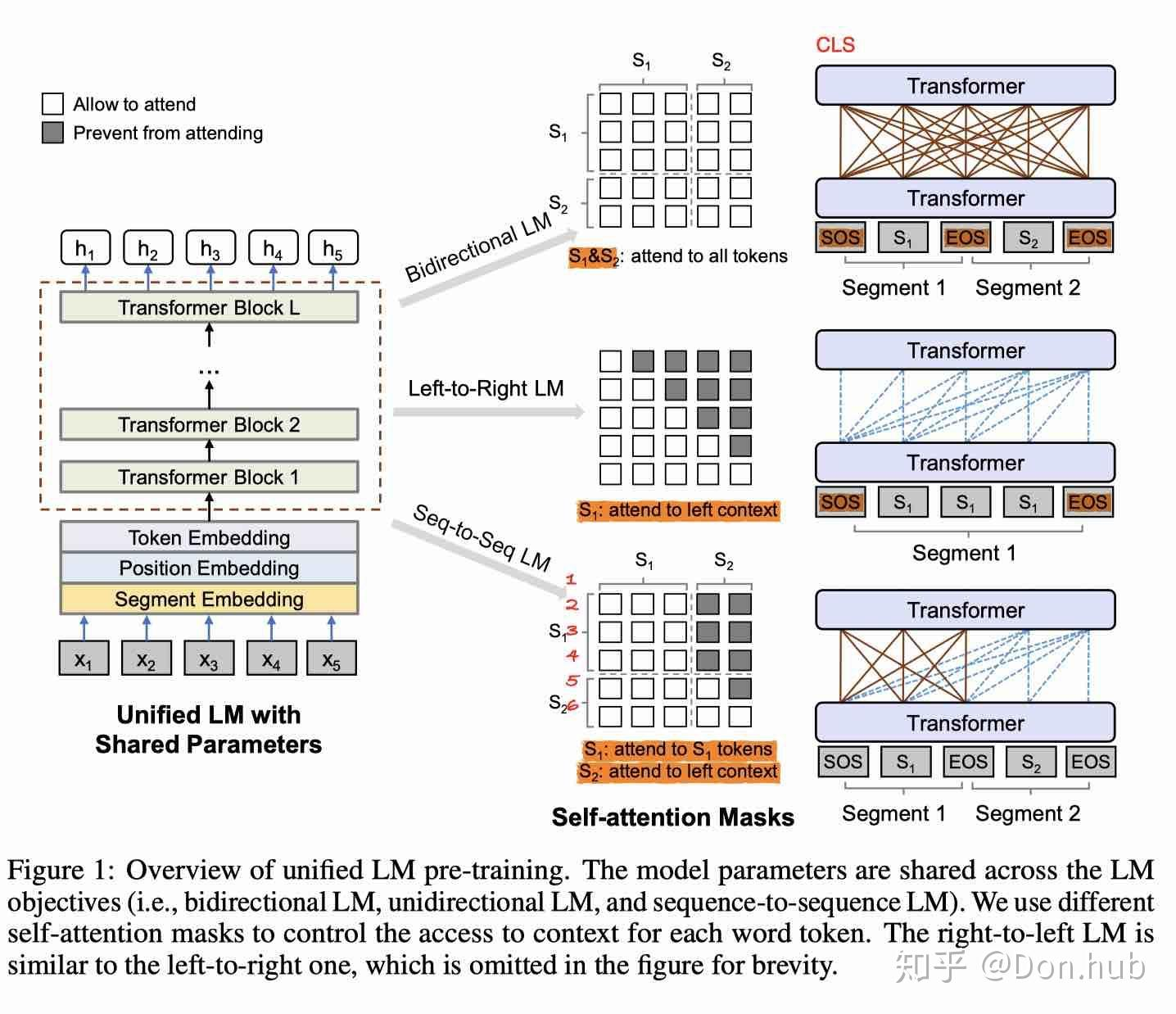• 预训练任务：自注意力矩阵分别如上图, 训练时编码和解码部分共享同一套参数

• 双向语言模型：输入序列由两个文本片段组成并由特殊标记[EOS]组成

• 单向语言模型：包括前后向

• 序列到序列语言模型：输入为条件文本片段和目标(待生成)文本片段，条件文本片段相互可见，目标文本片段仅历史和条件可见，也称为前缀语言模型(Prefix LM)

因此，在自回归生成中，目标序列和条件序列具备跨越的注意力机制，这是 BART 的 Encoder-Decoder 结构所不有的

• 下游任务精调

• 语言模型选择：语言理解类任务使用双向语言模型，文本生成类使用单向或者序列到序列
• 分类任务：与 BERT 类似，使用双向，将输入序列第一个标记[BOS]处的最后一层隐含层表示作为文本表示
• 生成任务：随机采样目标文本片段中的单词并掩码，精调学习目标是恢复这些被掩码的词。输入尾部的[EOS]也会被掩码，从而让模型学习到什么时候停止生成

III. T5

• 基本思想：统一所有任务为条件式文本生成任务，从而方便不同任务间的迁移学习和多任务学习，也不用为每个任务定义不同的模型结构和训练方法

• 使用同一套模型完成多个不同的条件式生成任务有两个关键因素

• 如何注入任务信息？自然语言描述或简短提示(Prompt)作为输入文本的前缀表示目标任务

例如：

翻译： translate English to German : xxx

语言可接受性判定：cola sentence : xxx

文本摘要：summarize : xxx

情感分类：sentiment : xxx

关于任务信息注入：

这是迁移学习中的常用技术，尤其是多任务学习以及元学习

常见的有：向量表示方法，自然语言描述方法，少量代表性样本方法等

• 模型容量：很大，得忍一下，最好的达到了约 110 亿个参数的规模

• 预训练任务：原则上编码器解码器、单向语言模型、序列到序列等生成模型结构都能用，但编码器-解码器结构表现相对较好

• 自监督预训练：类似于 BART 的文本填充任务，但是稍有不同如图：